# Quantum One Introduction So what is quantum mechanics

• Slides: 96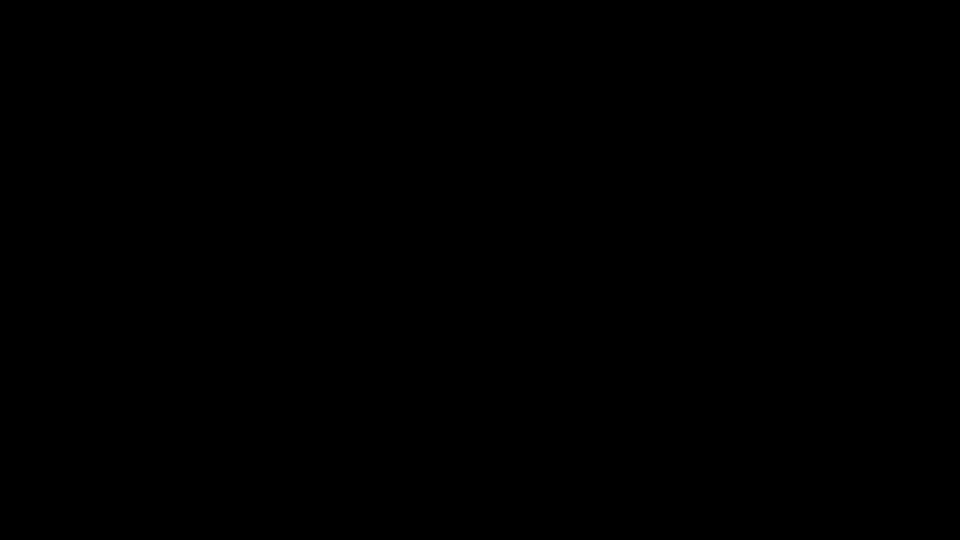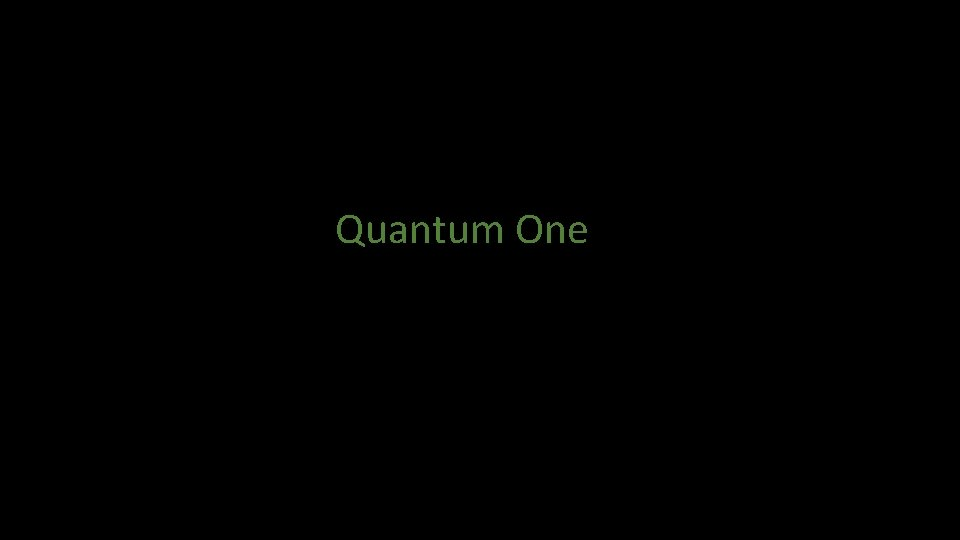Quantum One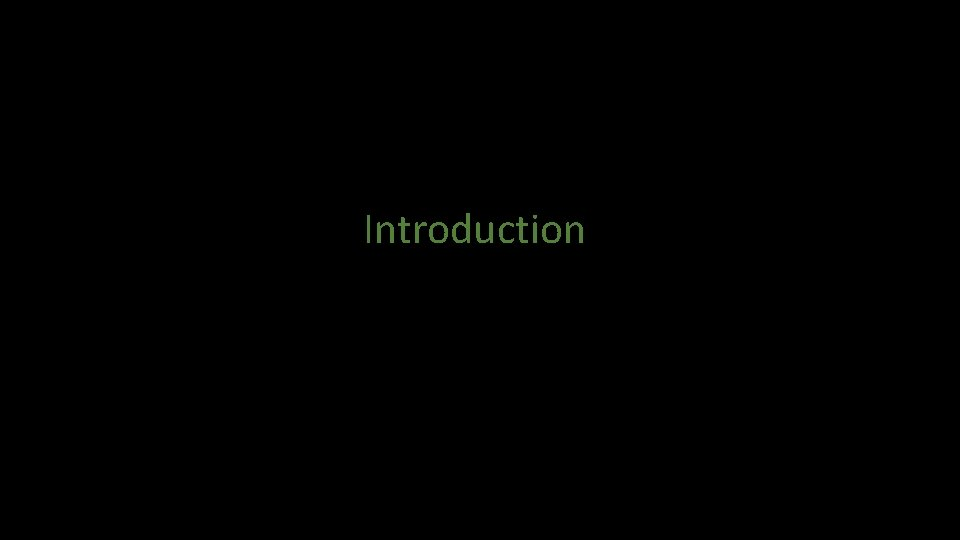Introduction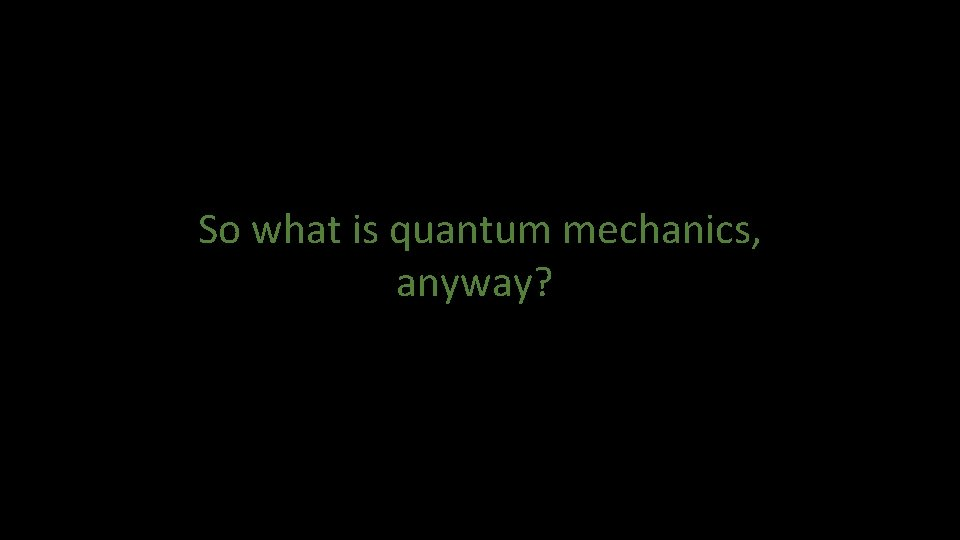So what is quantum mechanics, anyway?Greetings! I'm Dr. Paul Parris, instructor of the two semester graduate level course in Quantum Mechanics offered by the physics department at Missouri University of Science & Technology. This is the first lecture in the first semester of the course, which I refer to as Quantum One. This opening lecture provides a conceptual introduction to the main topic of the course, and begins by posing the simple question: So What is Quantum Mechanics, Anyway?Greetings! I'm Dr. Paul Parris, instructor of the two semester graduate level course in Quantum Mechanics offered by the physics department at Missouri University of Science & Technology. This is the first lecture in the first semester of the course, which I refer to as Quantum One. This opening lecture provides a conceptual introduction to the main topic of the course, and begins by posing the simple question: So What is Quantum Mechanics, Anyway?Greetings! I'm Dr. Paul Parris, instructor of the two semester graduate level course in Quantum Mechanics offered by the physics department at Missouri University of Science & Technology. This is the first lecture in the first semester of the course, which I refer to as Quantum One. This opening lecture provides a conceptual introduction to the main topic of the course, and begins by posing the simple question: So What is Quantum Mechanics, Anyway?Greetings! I'm Dr. Paul Parris, instructor of the two semester graduate level course in Quantum Mechanics offered by the physics department at Missouri University of Science & Technology. This is the first lecture in the first semester of the course, which I refer to as Quantum One. This opening lecture provides a conceptual introduction to the main topic of the course, and begins by posing the simple question: So What is Quantum Mechanics, Anyway?Greetings! I'm Dr. Paul Parris, instructor of the two semester graduate level course in Quantum Mechanics offered by the physics department at Missouri University of Science & Technology. This is the first lecture in the first semester of the course, which I refer to as Quantum One. This opening lecture provides a conceptual introduction to the main topic of the course, and begins by posing the simple question: So What is Quantum Mechanics, Anyway?So what is quantum mechanics? Evidently, it is some form of mechanics. You are probably familiar with, or have heard of other forms of mechanics…. There is, of course, the original, which for a long time was simply referred to as Mechanics but which these days is usually referred to as Classical Mechanics You will probably have noticed that Classical Mechanics seems to come in several different versions, like …So what is quantum mechanics? Evidently, it is some form of mechanics. You are probably familiar with, or have heard of other forms of mechanics…. There is, of course, the original, which for a long time was simply referred to as Mechanics but which these days is usually referred to as Classical Mechanics You will probably have noticed that Classical Mechanics seems to come in several different versions, like …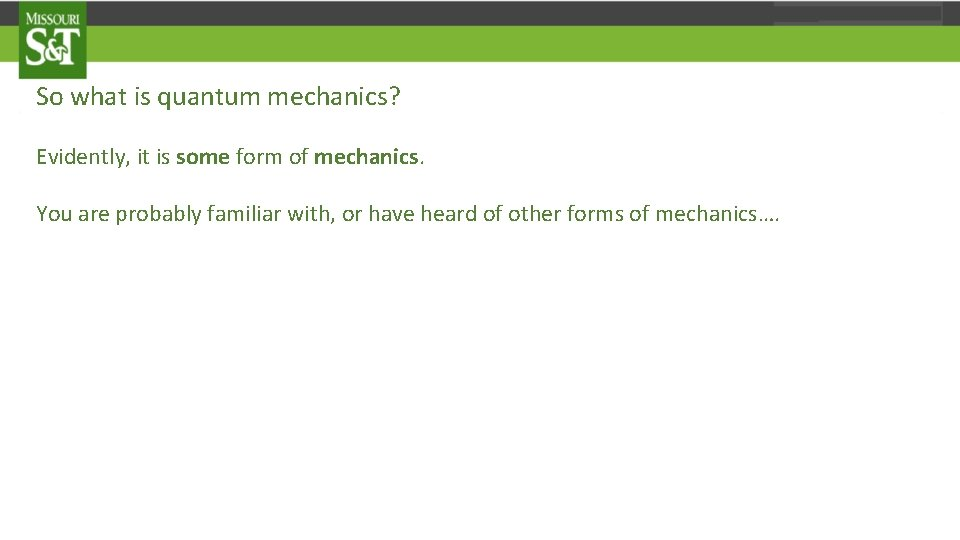So what is quantum mechanics? Evidently, it is some form of mechanics. You are probably familiar with, or have heard of other forms of mechanics…. There is, of course, the original, which for a long time was simply referred to as Mechanics but which these days is usually referred to as Classical Mechanics You will probably have noticed that Classical Mechanics seems to come in several different versions, like …So what is quantum mechanics? Evidently, it is some form of mechanics. You are probably familiar with, or have heard of other forms of mechanics…. There is, of course, the original, which for a long time was simply referred to as Mechanics but which these days is usually referred to as Classical Mechanics You will probably have noticed that Classical Mechanics seems to come in several different versions, like …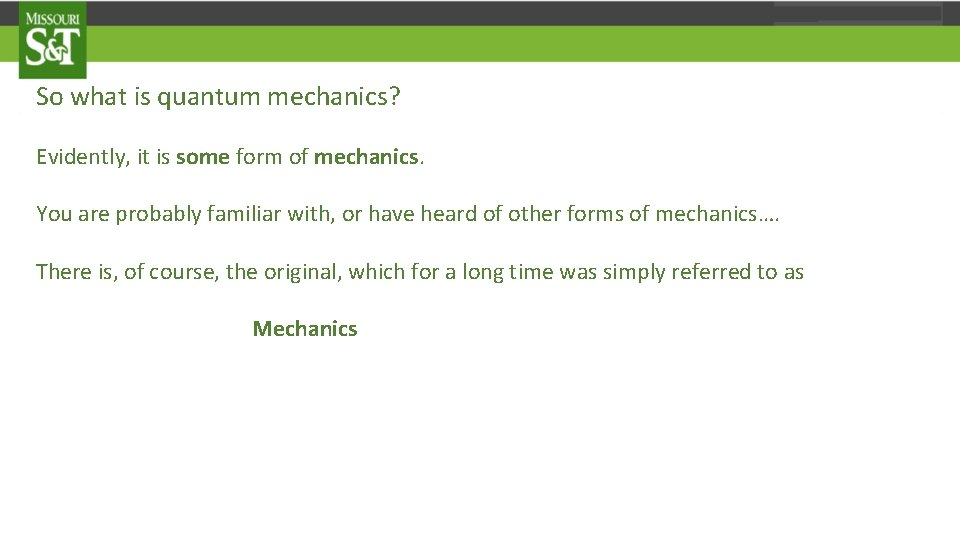So what is quantum mechanics? Evidently, it is some form of mechanics. You are probably familiar with, or have heard of other forms of mechanics…. There is, of course, the original, which for a long time was simply referred to as Mechanics but which these days is usually referred to as Classical Mechanics You will probably have noticed that Classical Mechanics seems to come in several different versions, like …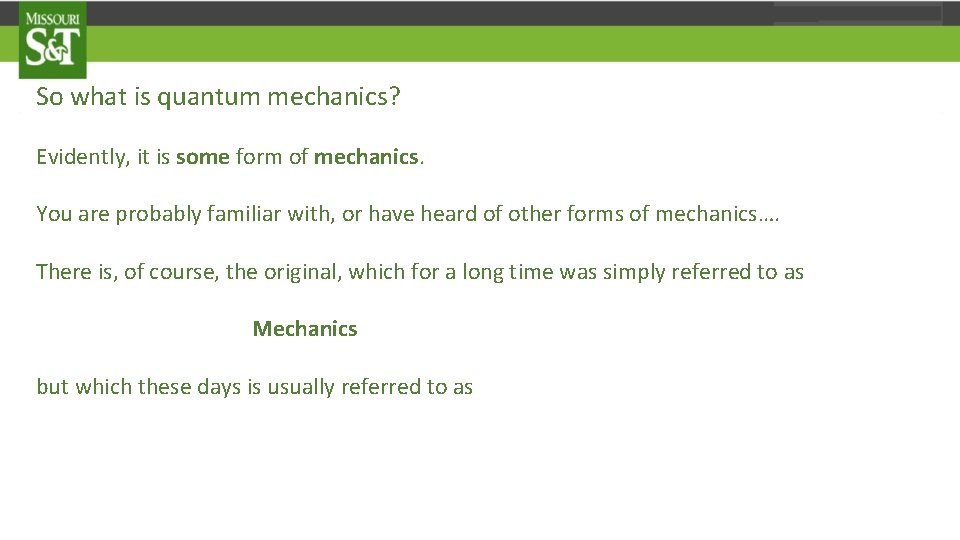So what is quantum mechanics? Evidently, it is some form of mechanics. You are probably familiar with, or have heard of other forms of mechanics…. There is, of course, the original, which for a long time was simply referred to as Mechanics but which these days is usually referred to as Classical Mechanics You will probably have noticed that Classical Mechanics seems to come in several different versions, like …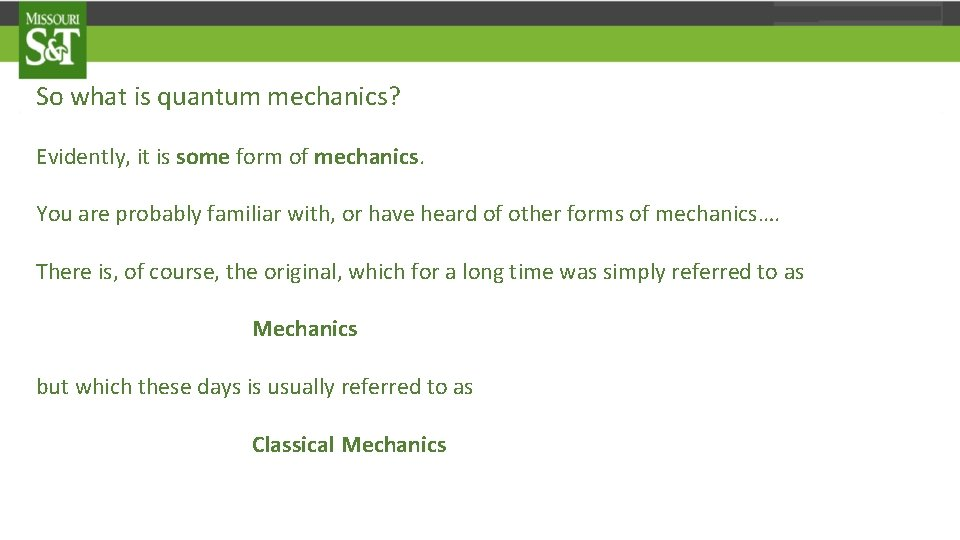So what is quantum mechanics? Evidently, it is some form of mechanics. You are probably familiar with, or have heard of other forms of mechanics…. There is, of course, the original, which for a long time was simply referred to as Mechanics but which these days is usually referred to as Classical Mechanics You will probably have noticed that Classical Mechanics seems to come in several different versions, like …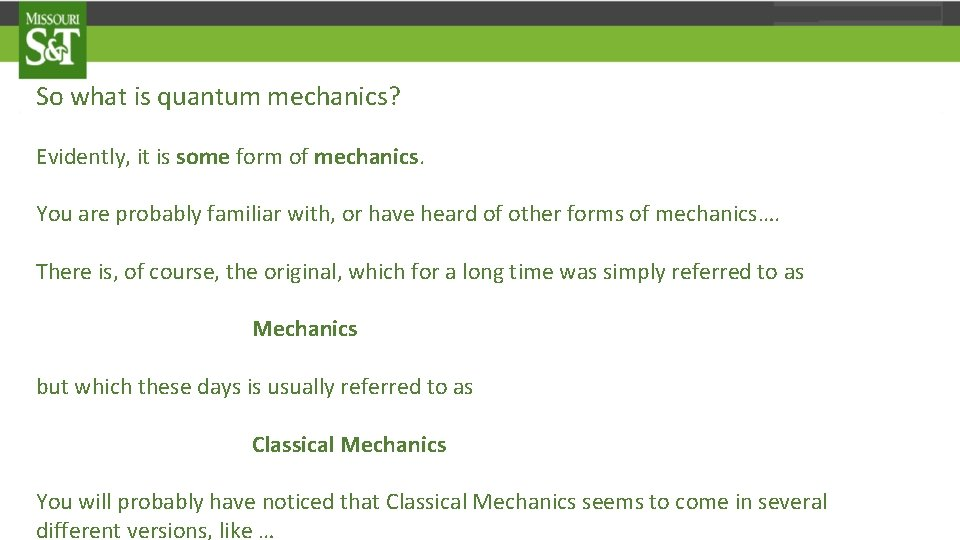So what is quantum mechanics? Evidently, it is some form of mechanics. You are probably familiar with, or have heard of other forms of mechanics…. There is, of course, the original, which for a long time was simply referred to as Mechanics but which these days is usually referred to as Classical Mechanics You will probably have noticed that Classical Mechanics seems to come in several different versions, like …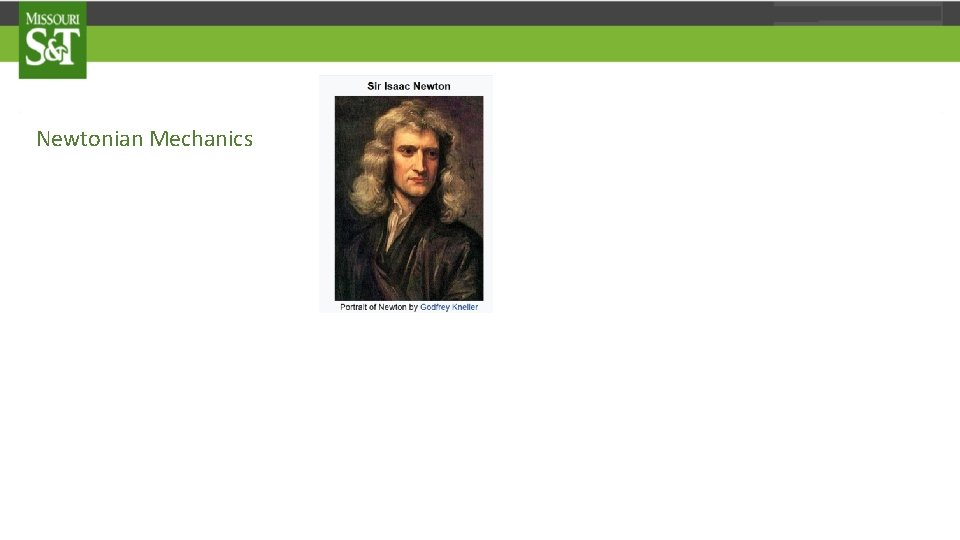Newtonian Mechanics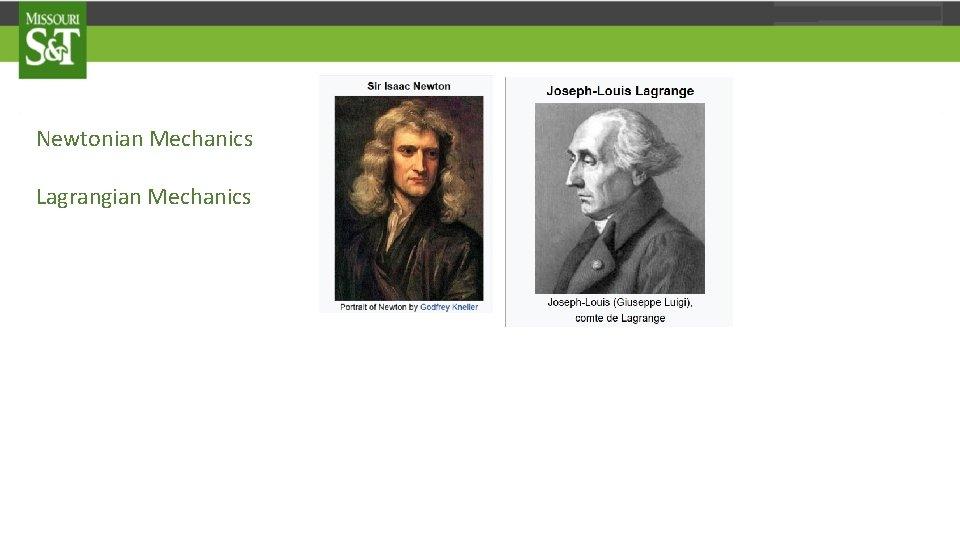Newtonian Mechanics Lagrangian Mechanics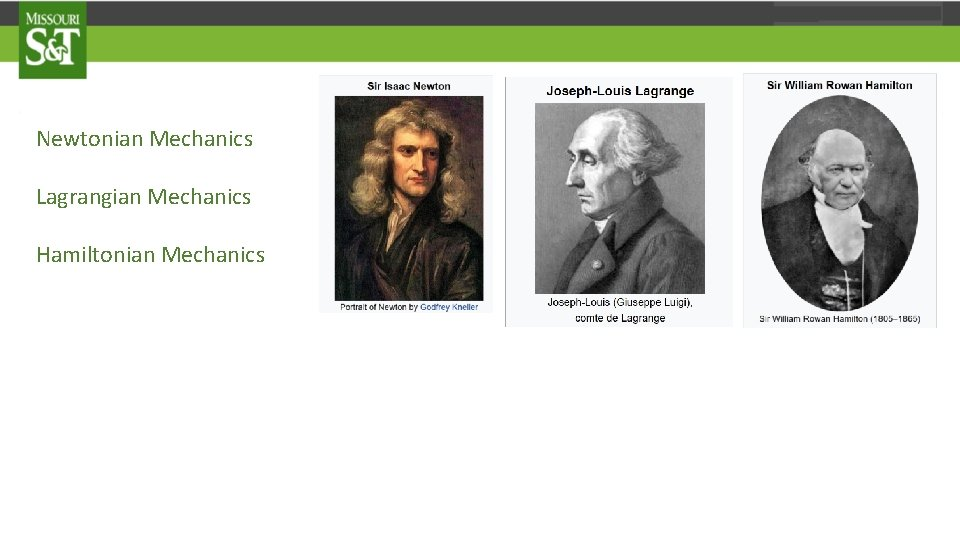Newtonian Mechanics Lagrangian Mechanics Hamiltonian Mechanics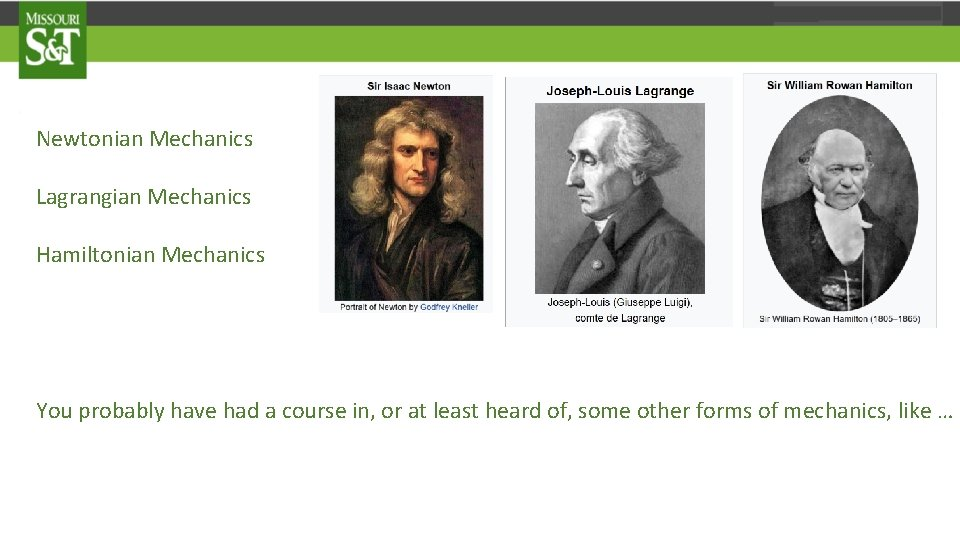Newtonian Mechanics Lagrangian Mechanics Hamiltonian Mechanics You probably have had a course in, or at least heard of, some other forms of mechanics, like …Statistical Mechanics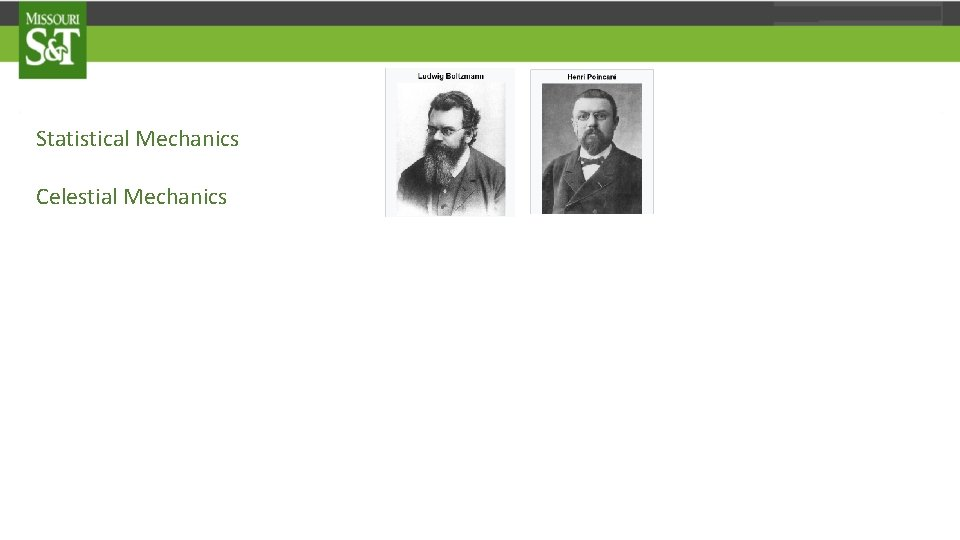Statistical Mechanics Celestial MechanicsStatistical Mechanics Celestial Mechanics Continuum Mechanics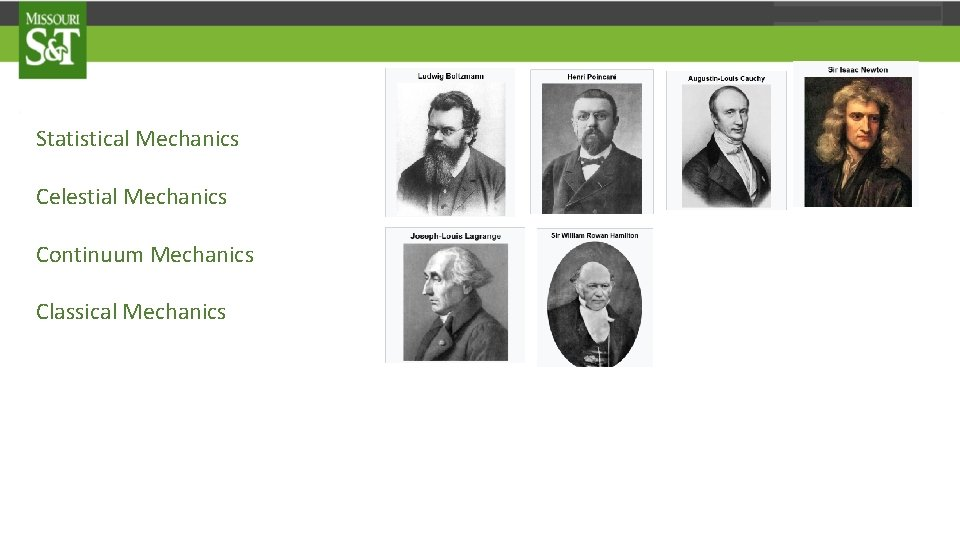Statistical Mechanics Celestial Mechanics Continuum Mechanics Classical Mechanics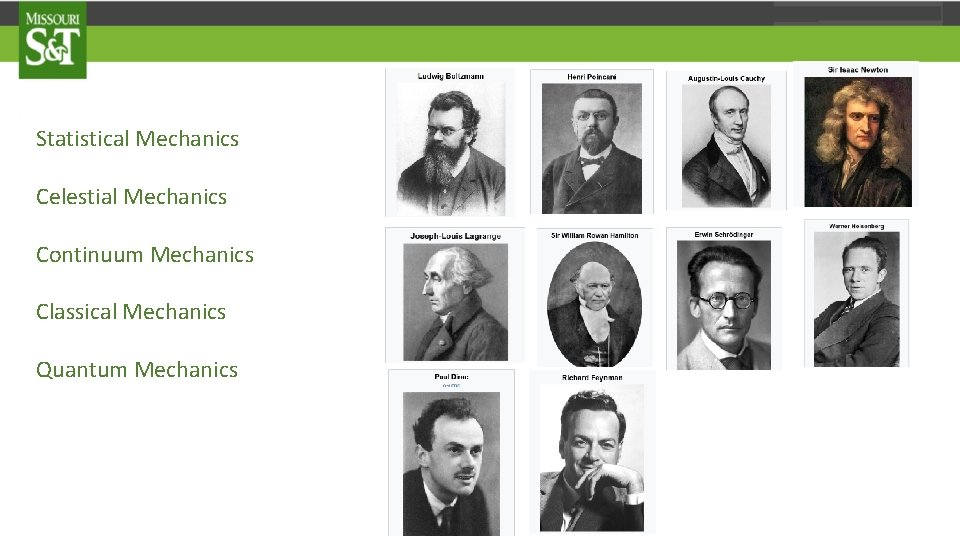Statistical Mechanics Celestial Mechanics Continuum Mechanics Classical Mechanics Quantum Mechanics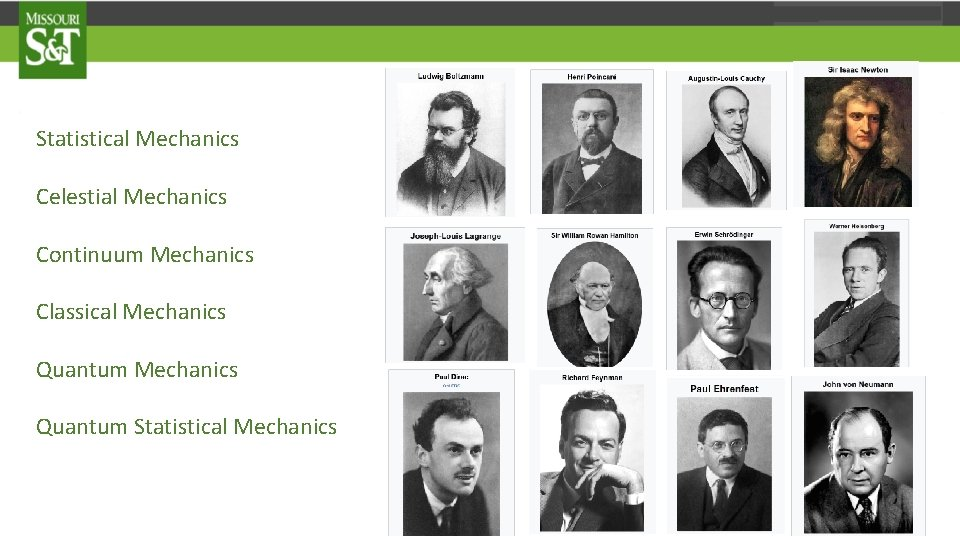Statistical Mechanics Celestial Mechanics Continuum Mechanics Classical Mechanics Quantum Statistical Mechanics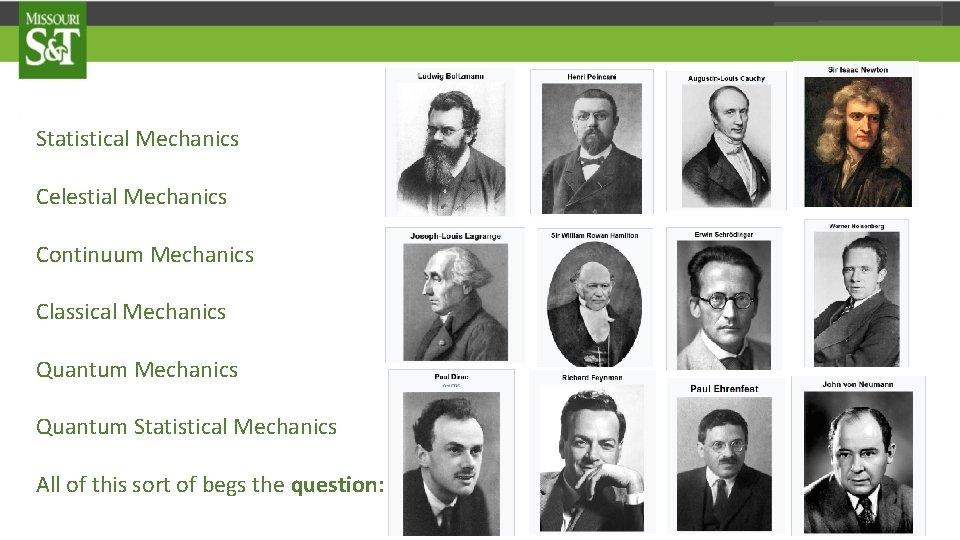Statistical Mechanics Celestial Mechanics Continuum Mechanics Classical Mechanics Quantum Statistical Mechanics All of this sort of begs the question: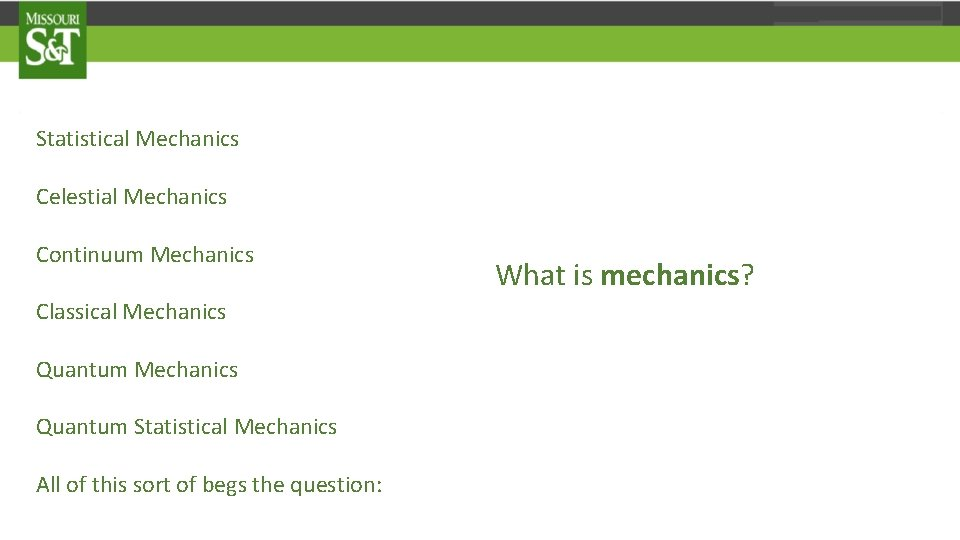Statistical Mechanics Celestial Mechanics Continuum Mechanics Classical Mechanics Quantum Statistical Mechanics All of this sort of begs the question: What is mechanics?Statistical Mechanics Celestial Mechanics Continuum Mechanics Classical Mechanics Quantum Statistical Mechanics I would assert, that in each of these different forms of mechanics, the practitioners involved have two primary goals: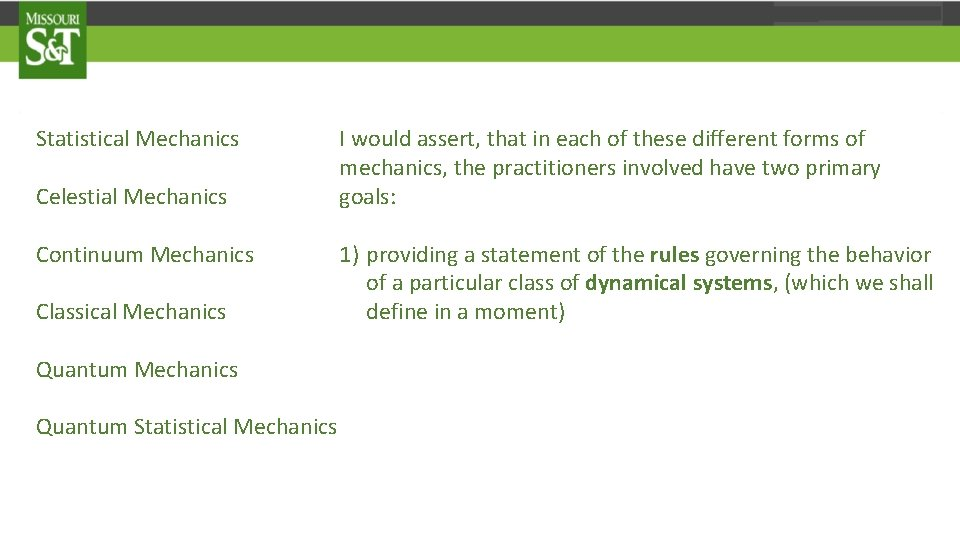Statistical Mechanics Celestial Mechanics Continuum Mechanics Classical Mechanics Quantum Statistical Mechanics I would assert, that in each of these different forms of mechanics, the practitioners involved have two primary goals: 1) providing a statement of the rules governing the behavior of a particular class of dynamical systems, (which we shall define in a moment)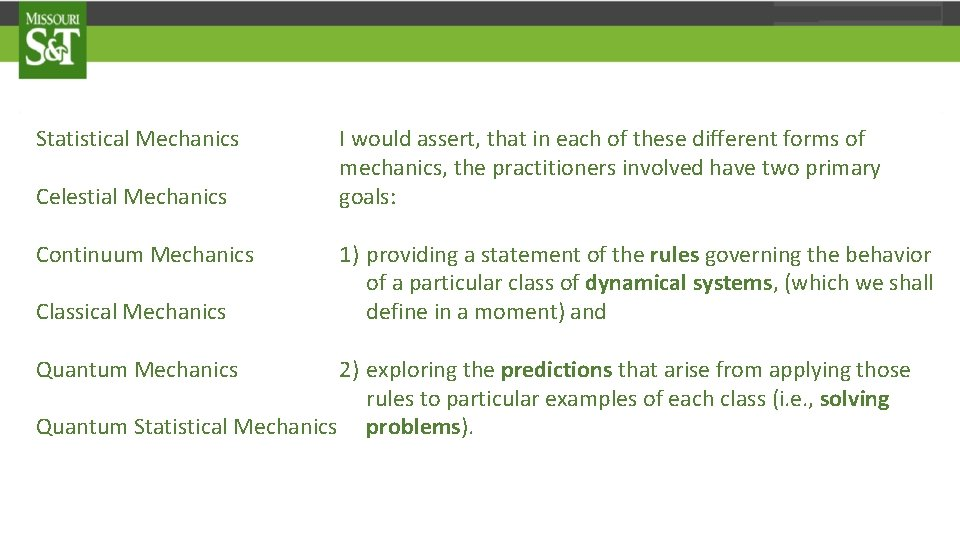Statistical Mechanics Celestial Mechanics Continuum Mechanics Classical Mechanics I would assert, that in each of these different forms of mechanics, the practitioners involved have two primary goals: 1) providing a statement of the rules governing the behavior of a particular class of dynamical systems, (which we shall define in a moment) and 2) exploring the predictions that arise from applying those rules to particular examples of each class (i. e. , solving Quantum Statistical Mechanics problems). Quantum Mechanics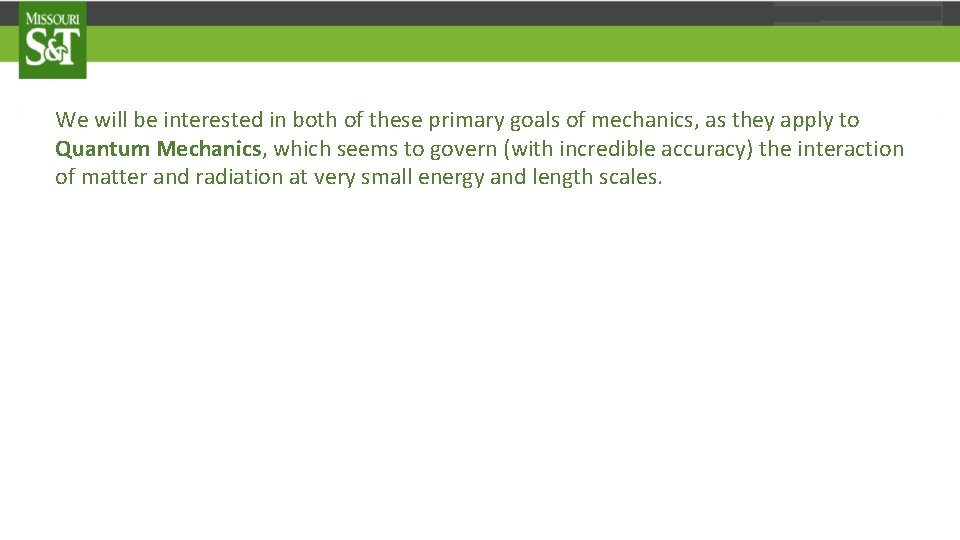We will be interested in both of these primary goals of mechanics, as they apply to Quantum Mechanics, which seems to govern (with incredible accuracy) the interaction of matter and radiation at very small energy and length scales.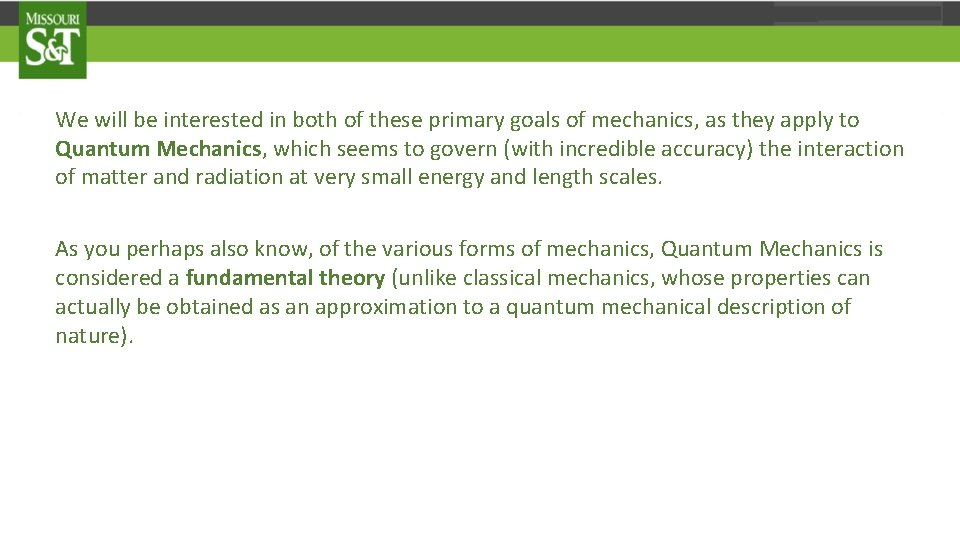We will be interested in both of these primary goals of mechanics, as they apply to Quantum Mechanics, which seems to govern (with incredible accuracy) the interaction of matter and radiation at very small energy and length scales. As you perhaps also know, of the various forms of mechanics, Quantum Mechanics is considered a fundamental theory (unlike classical mechanics, whose properties can actually be obtained as an approximation to a quantum mechanical description of nature).We will be interested in both of these primary goals of mechanics, as they apply to Quantum Mechanics, which seems to govern (with incredible accuracy) the interaction of matter and radiation at very small energy and length scales. As you perhaps also know, of the various forms of mechanics, Quantum Mechanics is considered a fundamental theory (unlike classical mechanics, whose properties can actually be obtained as an approximation to a quantum mechanical description of nature). Indeed, if a unique Theory Of Everything is ever successfully developed, there is little doubt that it will ultimately be some kind of Quantum Mechanical theory.Thus, we will be interested in:Thus, we will be interested in: 1) providing a statement of the rules governing the behavior of Quantum Mechanical systems, andThus, we will be interested in: 1) providing a statement of the rules governing the behavior of Quantum Mechanical systems, and 2) exploring the predictions that arise from applying those rules to particular examples of such systems, like atoms and fundamental particles.Thus, we will be interested in: 1) providing a statement of the rules governing the behavior of Quantum Mechanical systems, and 2) exploring the predictions that arise from applying those rules to particular examples of such systems, like atoms and fundamental particles. But we cannot explore the predictions that arise from the rules, until we know what the rules are. Stating these rules clearly and succinctly will therefore concern us a great deal.Thus, we will be interested in: 1) providing a statement of the rules governing the behavior of Quantum Mechanical systems, and 2) Exploring the predictions that arise from applying those rules to particular examples of such systems, like atoms and fundamental particles. But we cannot explore the predictions that arise from the rules, until we know what the rules are. Stating these rules clearly and succinctly will therefore concern us a great deal. Although not the only way to do it, an efficient way of doing this for a given form of mechanics is by stating the rules in terms of postulates, the number and form of which follow essentially from the definition of the term dynamical system.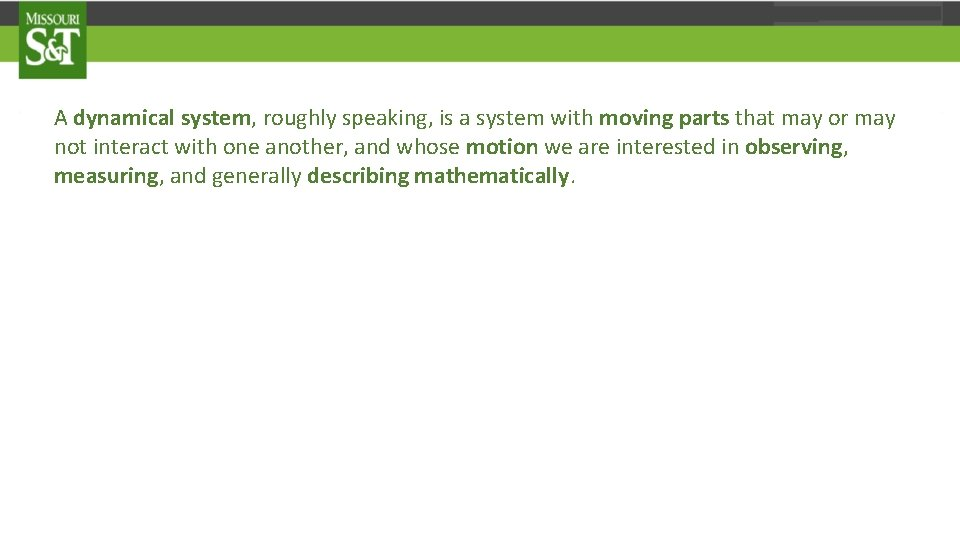A dynamical system, roughly speaking, is a system with moving parts that may or may not interact with one another, and whose motion we are interested in observing, measuring, and generally describing mathematically.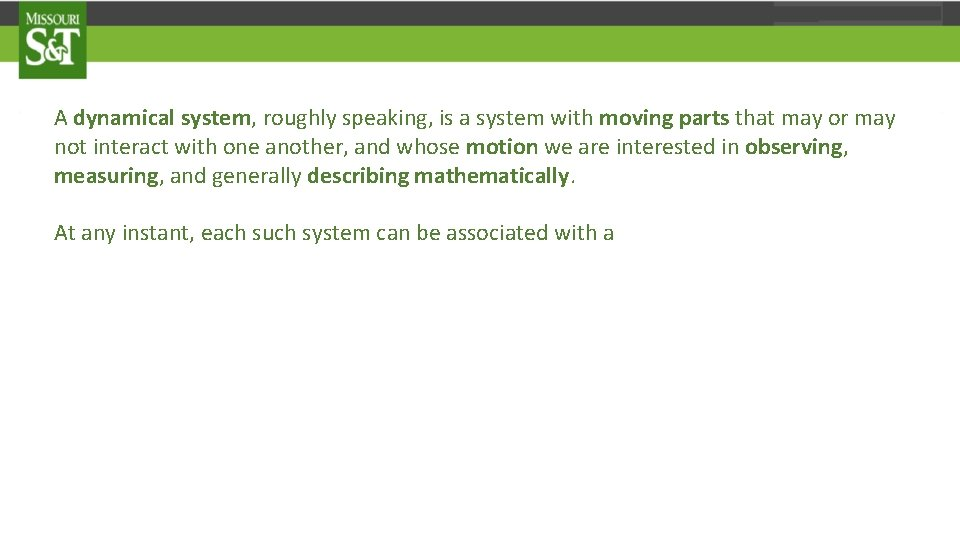A dynamical system, roughly speaking, is a system with moving parts that may or may not interact with one another, and whose motion we are interested in observing, measuring, and generally describing mathematically. At any instant, each such system can be associated with aA dynamical system, roughly speaking, is a system with moving parts that may or may not interact with one another, and whose motion we are interested in observing, measuring, and generally describing mathematically. At any instant, each such system can be associated with a dynamical stateA dynamical system, roughly speaking, is a system with moving parts that may or may not interact with one another, and whose motion we are interested in observing, measuring, and generally describing mathematically. At any instant, each such system can be associated with a dynamical state which describes itsA dynamical system, roughly speaking, is a system with moving parts that may or may not interact with one another, and whose motion we are interested in observing, measuring, and generally describing mathematically. At any instant, each such system can be associated with a dynamical state which describes its configuration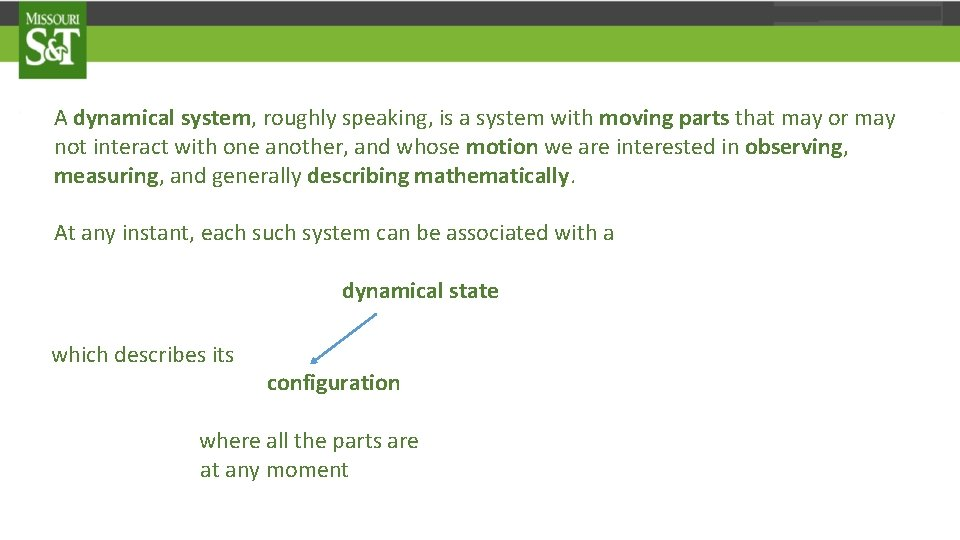A dynamical system, roughly speaking, is a system with moving parts that may or may not interact with one another, and whose motion we are interested in observing, measuring, and generally describing mathematically. At any instant, each such system can be associated with a dynamical state which describes its configuration where all the parts are at any moment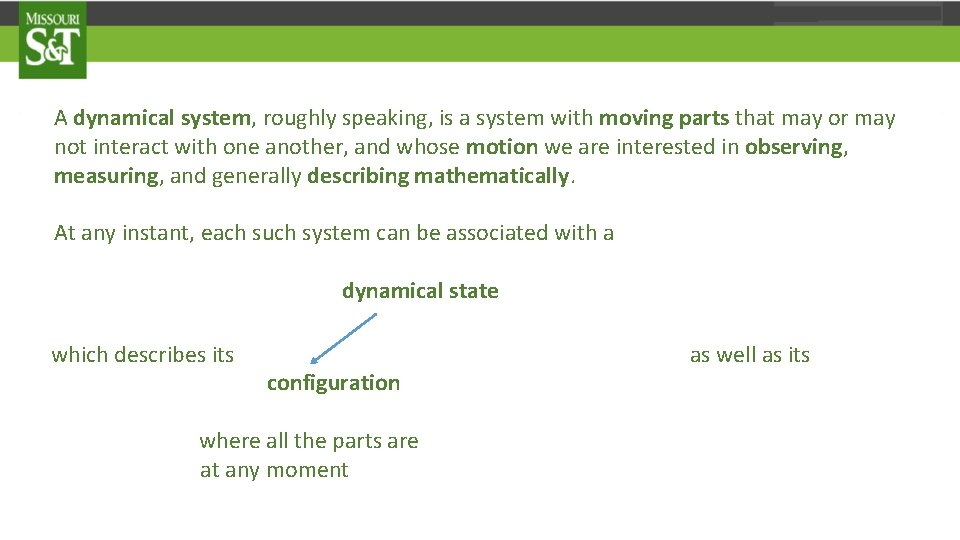A dynamical system, roughly speaking, is a system with moving parts that may or may not interact with one another, and whose motion we are interested in observing, measuring, and generally describing mathematically. At any instant, each such system can be associated with a dynamical state which describes its configuration where all the parts are at any moment as well as itsA dynamical system, roughly speaking, is a system with moving parts that may or may not interact with one another, and whose motion we are interested in observing, measuring, and generally describing mathematically. At any instant, each such system can be associated with a dynamical state which describes its configuration where all the parts are at any moment state of motion as well as its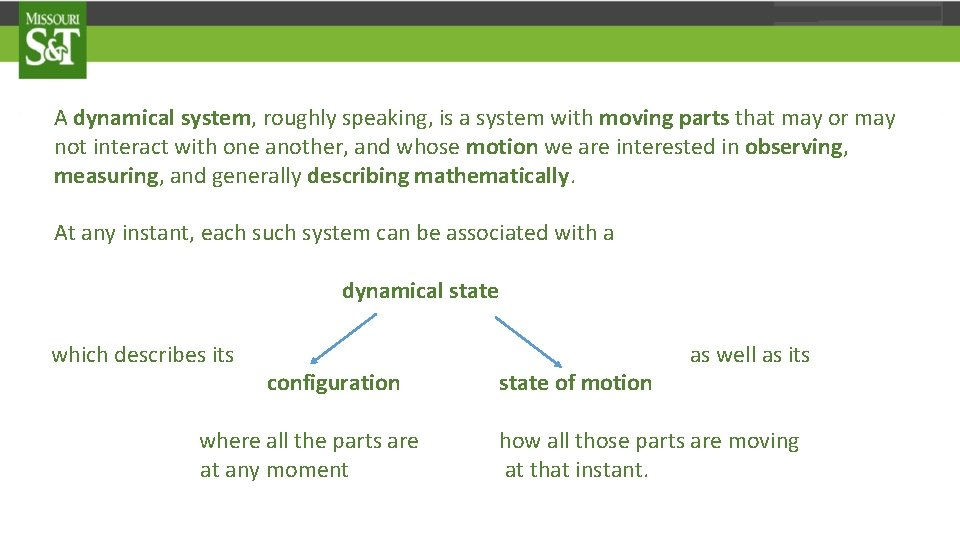A dynamical system, roughly speaking, is a system with moving parts that may or may not interact with one another, and whose motion we are interested in observing, measuring, and generally describing mathematically. At any instant, each such system can be associated with a dynamical state which describes its configuration where all the parts are at any moment state of motion as well as its how all those parts are moving at that instant.Postulates of Mechanics The postulates that define each form of mechanics, either implicitly or explicitly, provide important information regarding the dynamical systems to which they apply. In particular, the postulates of mechanics describe: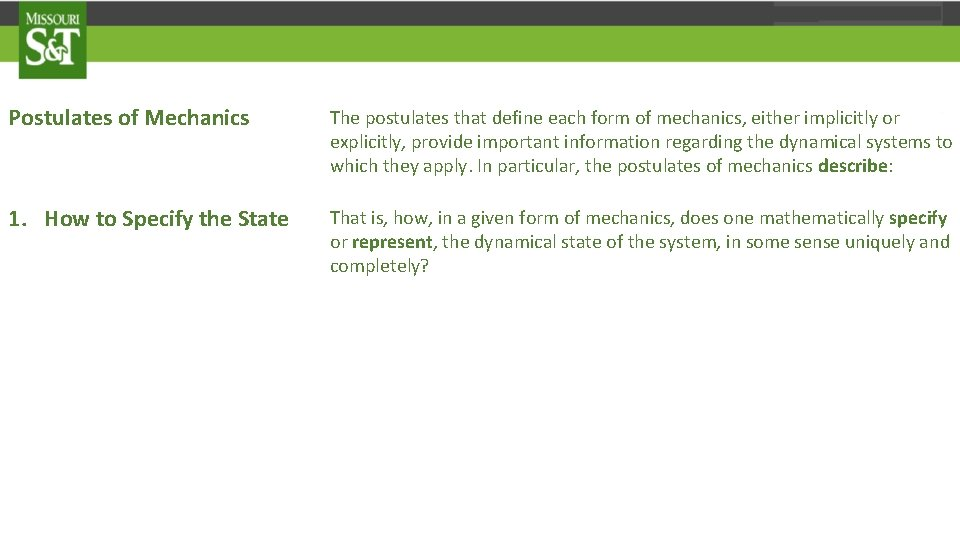Postulates of Mechanics The postulates that define each form of mechanics, either implicitly or explicitly, provide important information regarding the dynamical systems to which they apply. In particular, the postulates of mechanics describe: 1. How to Specify the State That is, how, in a given form of mechanics, does one mathematically specify or represent, the dynamical state of the system, in some sense uniquely and completely?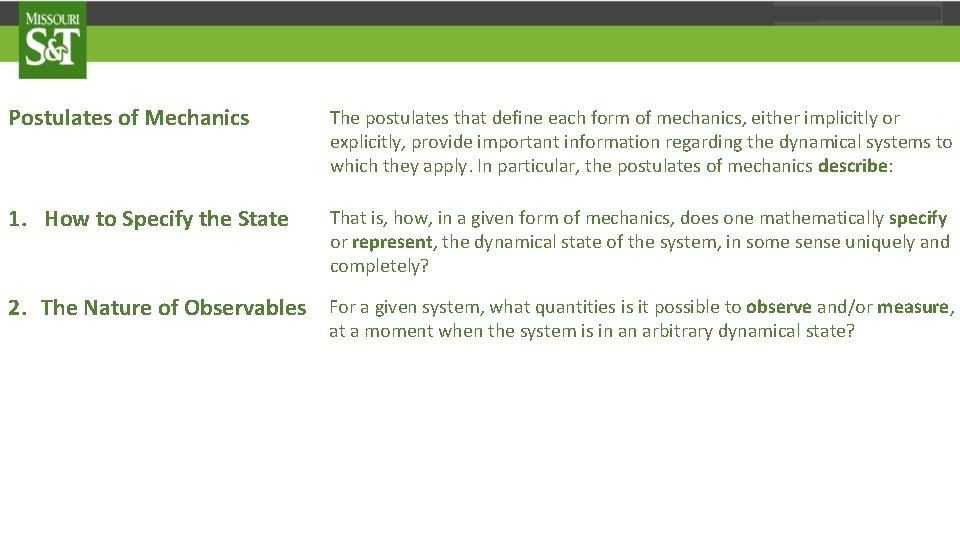Postulates of Mechanics The postulates that define each form of mechanics, either implicitly or explicitly, provide important information regarding the dynamical systems to which they apply. In particular, the postulates of mechanics describe: 1. How to Specify the State That is, how, in a given form of mechanics, does one mathematically specify or represent, the dynamical state of the system, in some sense uniquely and completely? 2. The Nature of Observables For a given system, what quantities is it possible to observe and/or measure, at a moment when the system is in an arbitrary dynamical state?Postulates of Mechanics The postulates that define each form of mechanics, either implicitly or explicitly, provide important information regarding the dynamical systems to which they apply. In particular, the postulates of mechanics describe: 1. How to Specify the State That, is how, in a given form of mechanics, does one mathematically specify or represent, the dynamical state of the system, in some sense uniquely and completely? 2. The Nature of Observables For a given system, what quantities is it possible to observe and/or measure, at a moment when the system is in an arbitrary dynamical state? 3. The Measurement Process What happens when an ideal measurement is made of an arbitrary observable of the system, at a moment when the system is in an arbitrary dynamical state? What values can be obtained, and what happens to the dynamical state itself as a result of the measurement process?Postulates of Mechanics The postulates that define each form of mechanics, either implicitly or explicitly, provide important information regarding the dynamical systems to which they apply. In particular, the postulates of mechanics describe: 1. How to Specify the State That, is how, in a given form of mechanics, does one mathematically specify or represent, the dynamical state of the system, in some sense uniquely and completely? 2. The Nature of Observables For a given system, what quantities is it possible to observe and/or measure, at a moment when the system is in an arbitrary dynamical state? 3. The Measurement Process What happens when an ideal measurement is made of an arbitrary observable of the system, at a moment when the system is in an arbitrary dynamical state? What values can be obtained, and what happens to the dynamical state itself as a result of the measurement process? 4. Evolution How does the system pass from one dynamical state to another, i. e. , what are the equations of motion?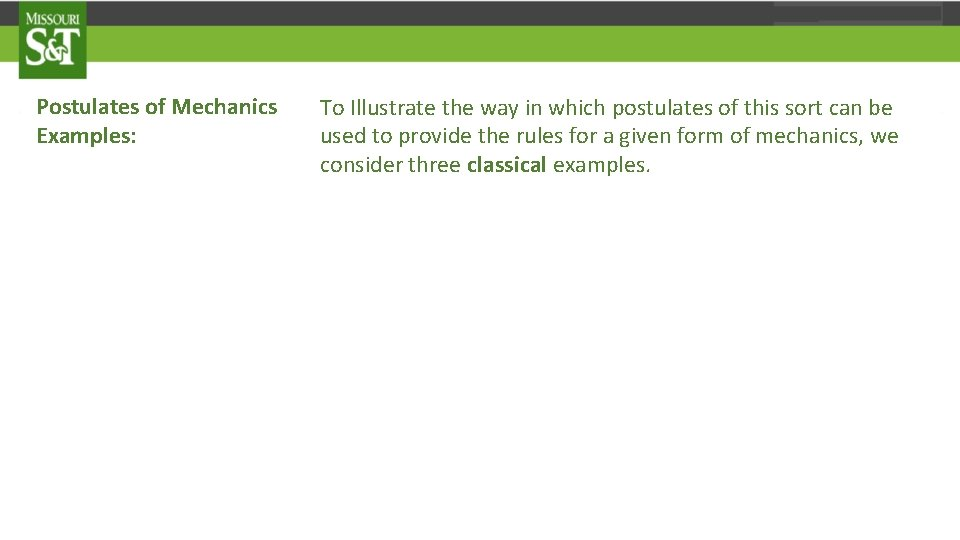Postulates of Mechanics Examples: To Illustrate the way in which postulates of this sort can be used to provide the rules for a given form of mechanics, we consider three classical examples.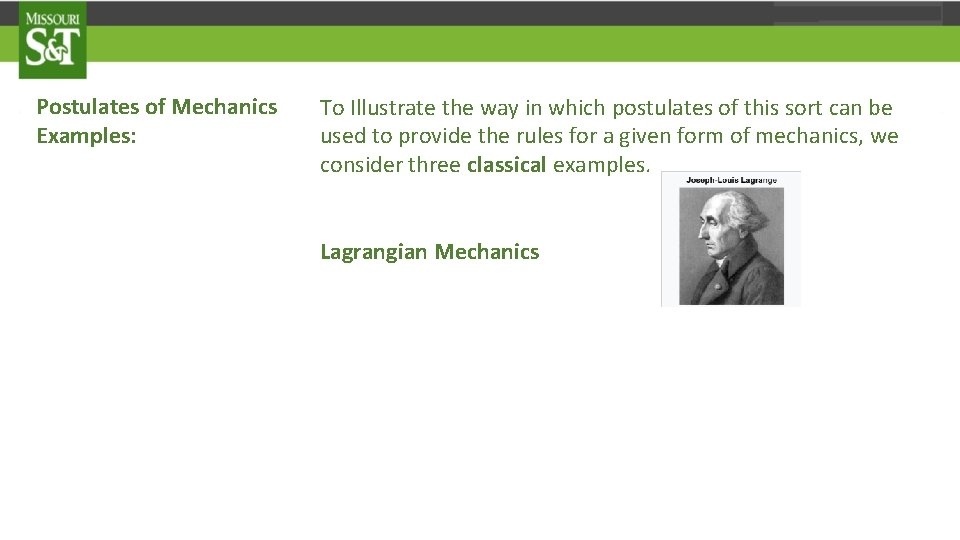Postulates of Mechanics Examples: To Illustrate the way in which postulates of this sort can be used to provide the rules for a given form of mechanics, we consider three classical examples. Lagrangian Mechanics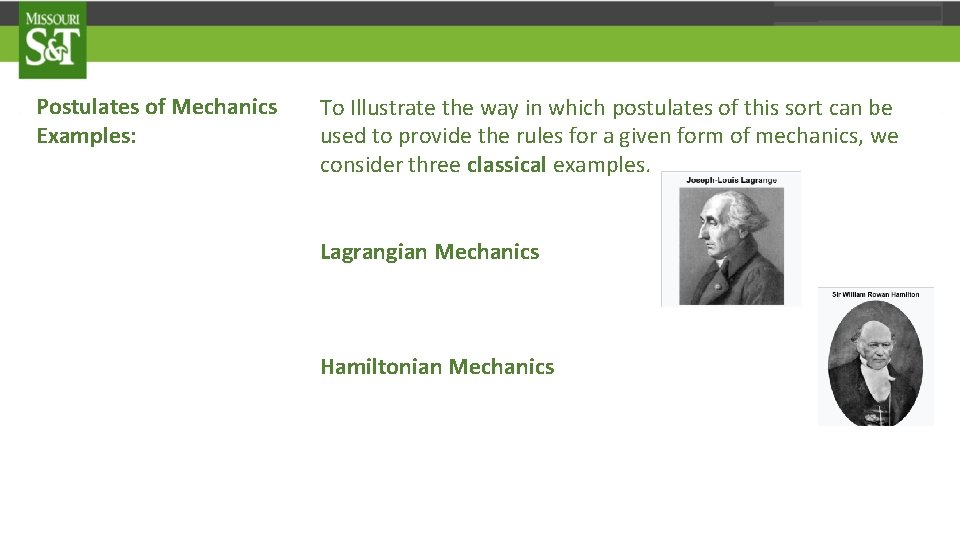Postulates of Mechanics Examples: To Illustrate the way in which postulates of this sort can be used to provide the rules for a given form of mechanics, we consider three classical examples. Lagrangian Mechanics Hamiltonian MechanicsPostulates of Mechanics Examples: To Illustrate the way in which postulates of this sort can be used to provide the rules for a given form of mechanics, we consider three classical examples. Lagrangian Mechanics Hamiltonian Mechanics Classical Statistical Mechanics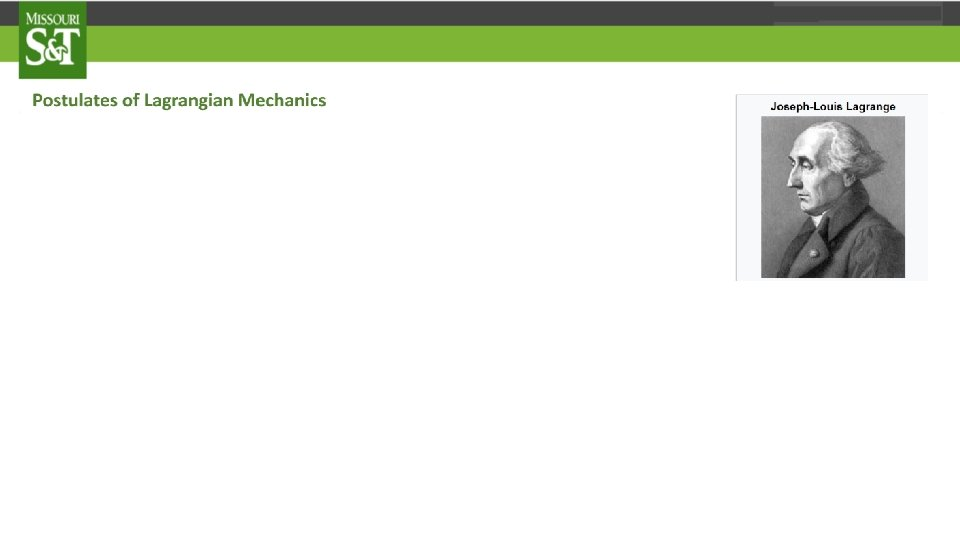q 3 q(t) . . q(t) q 1 q 2 configuration space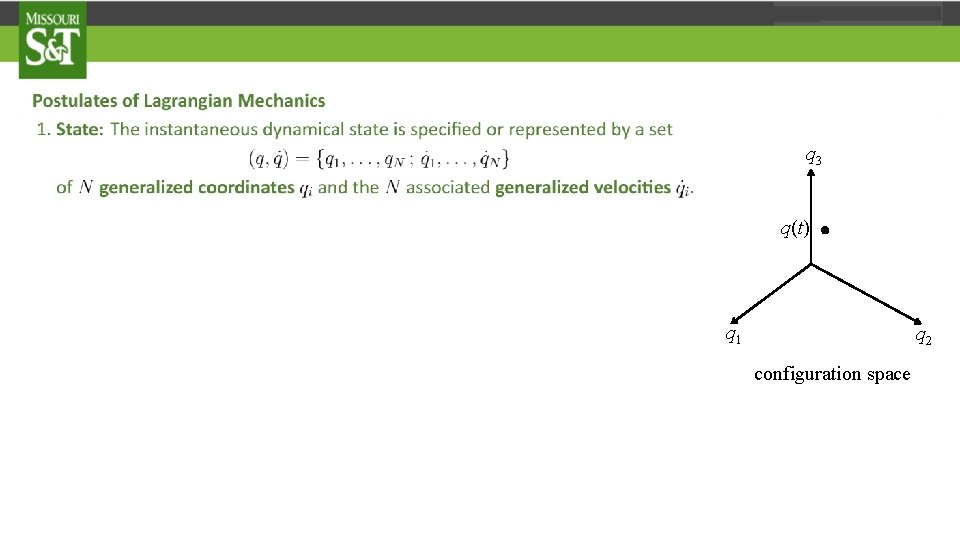q 3 q(t) . q 1 q 2 configuration space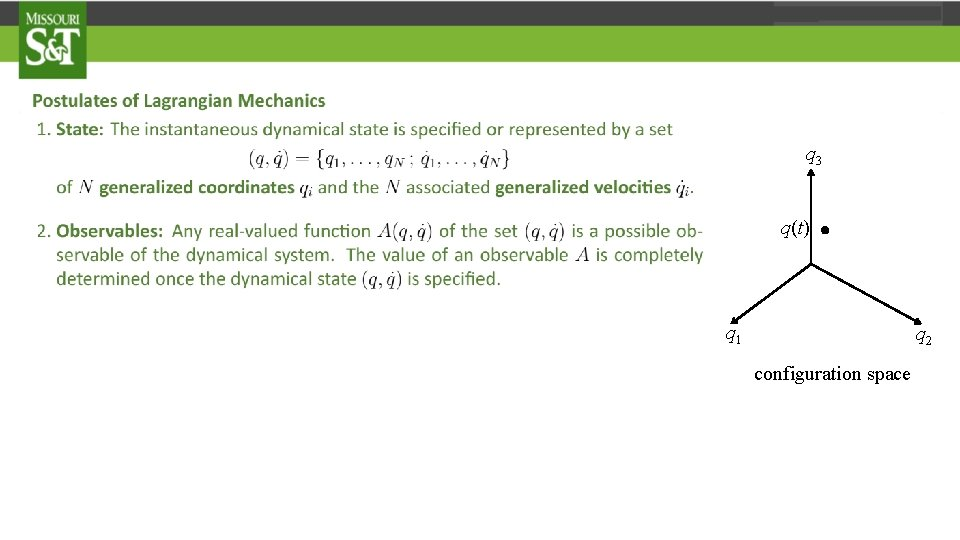q 3 q(t) . q 1 q 2 configuration space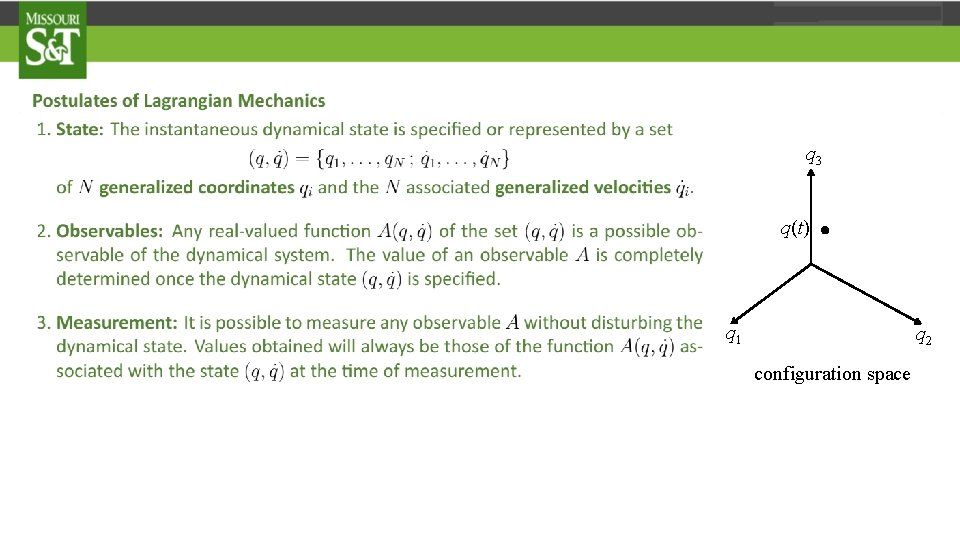q 3 q(t) . q 1 q 2 configuration space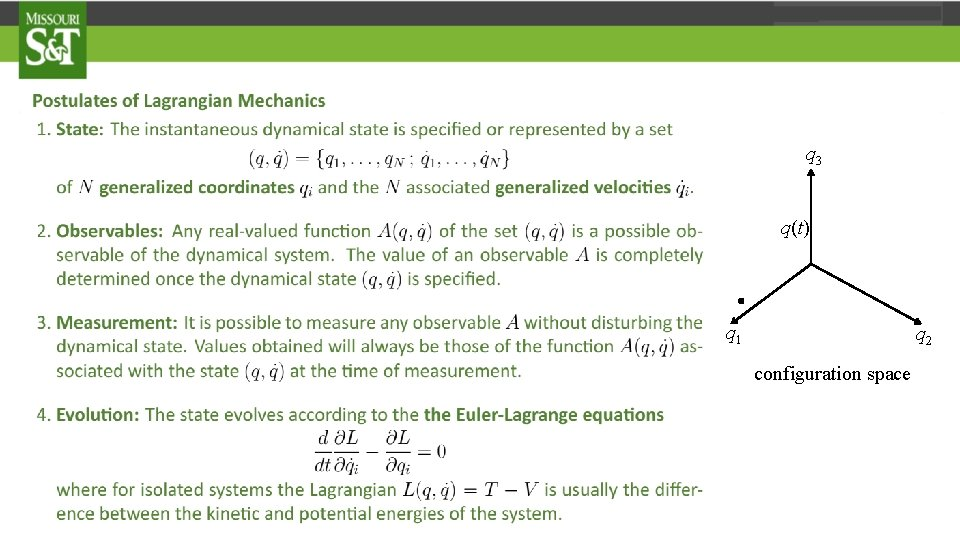q 3 q(t) . q 1 q 2 configuration space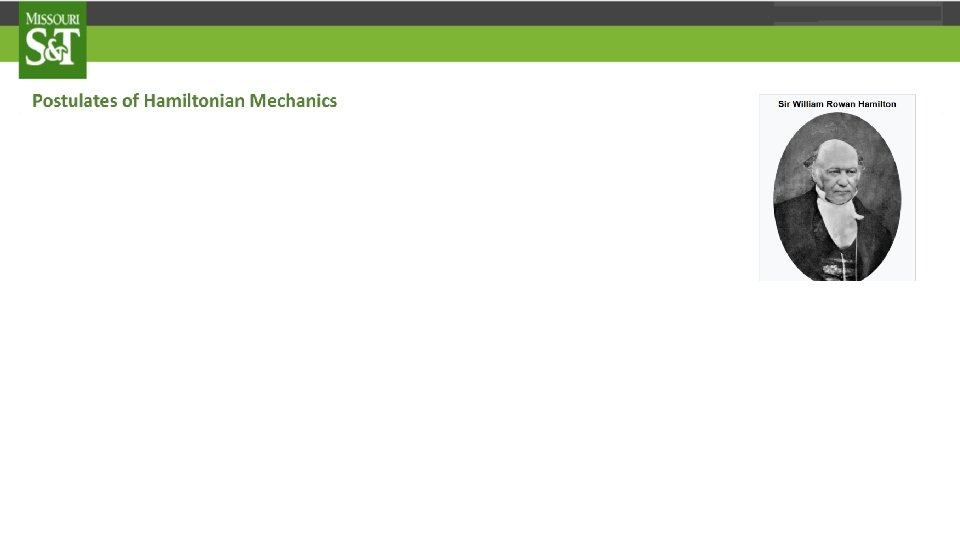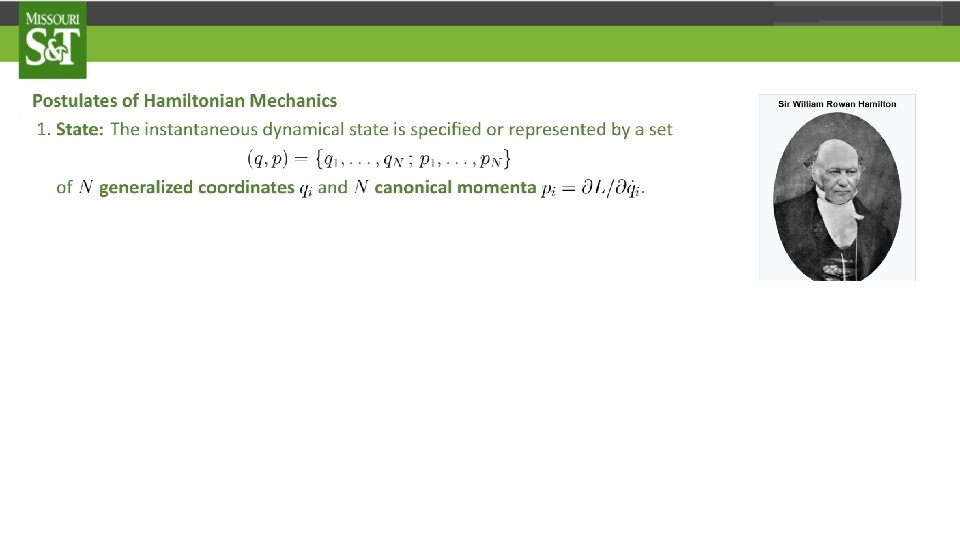p 1 q(t), p(t) . q 2 q 1 phase space (q, p)p 1 q(t), p(t) . q 2 q 1 phase space (q, p)p 1 q(t), p(t) . q 2 q 1 phase space (q, p)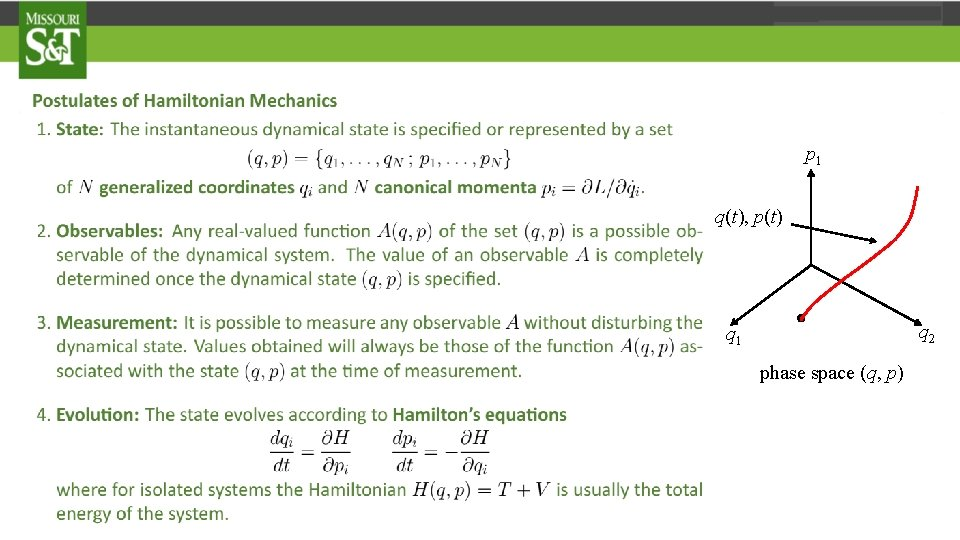p 1 q(t), p(t) q 1 . phase space (q, p) q 2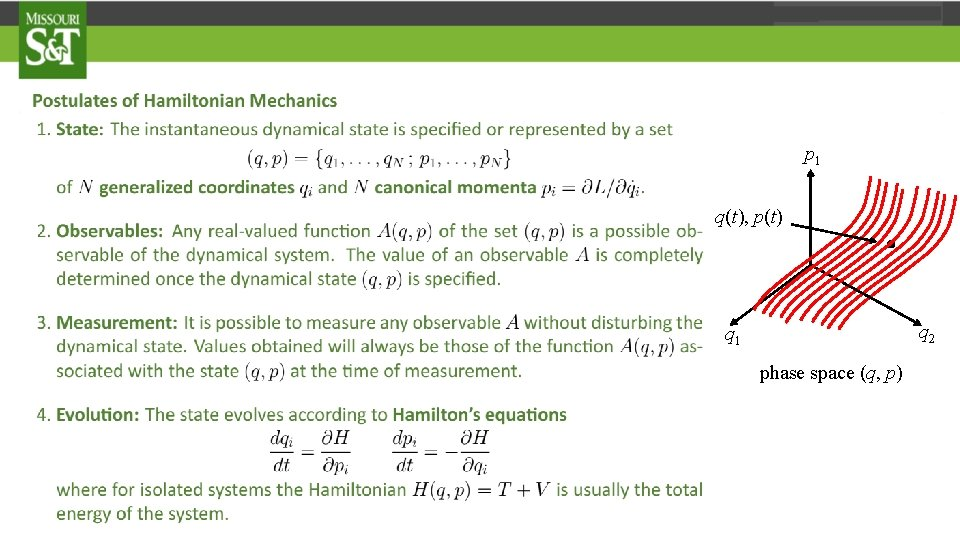p 1 q(t), p(t) . q 2 q 1 phase space (q, p)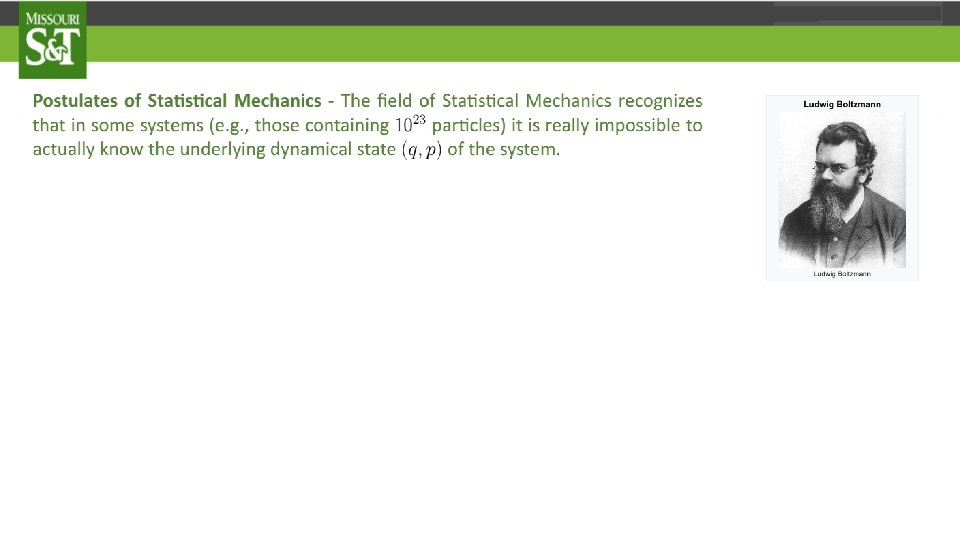p 1 . q, p q 1 phase space (q, p) q 2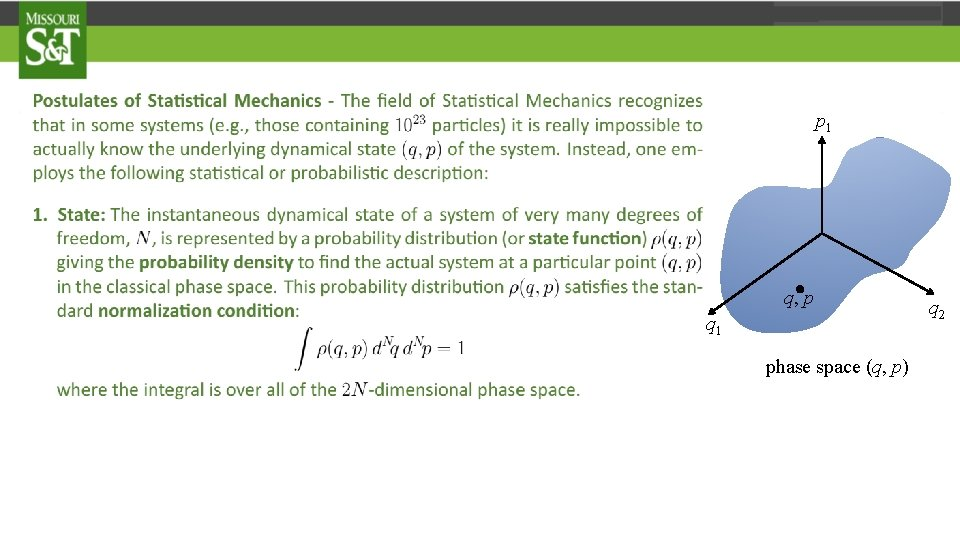p 1 . q, p q 1 phase space (q, p) q 2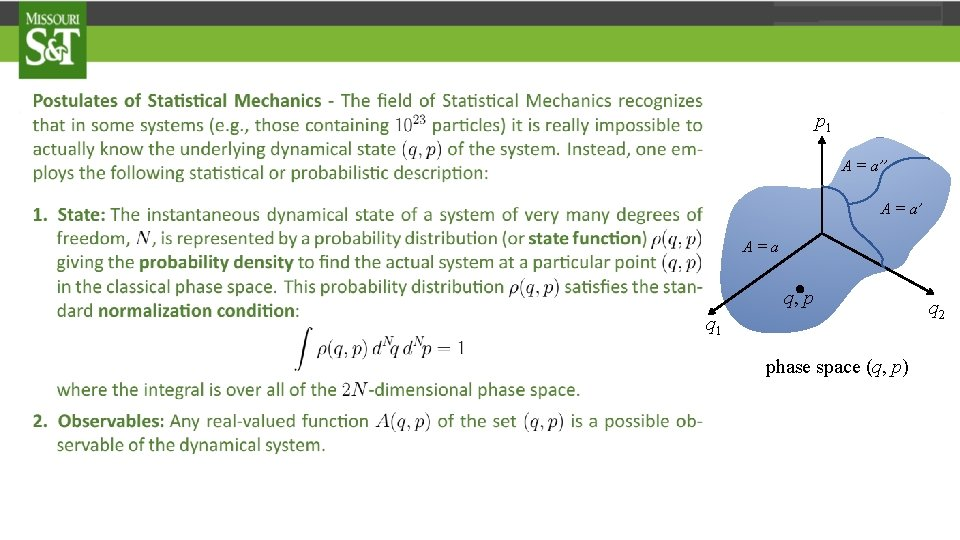p 1 A = a’’ A = a’ A=a . q, p q 1 phase space (q, p) q 2p 1 A = a’’ A = a’ A=a . q, p q 1 phase space (q, p) q 2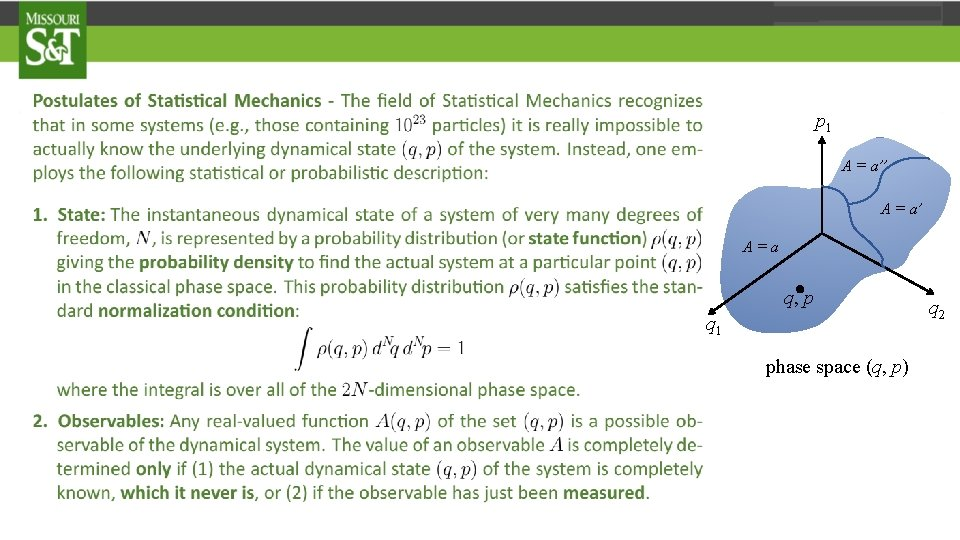p 1 A = a’’ A = a’ A=a . q, p q 1 phase space (q, p) q 2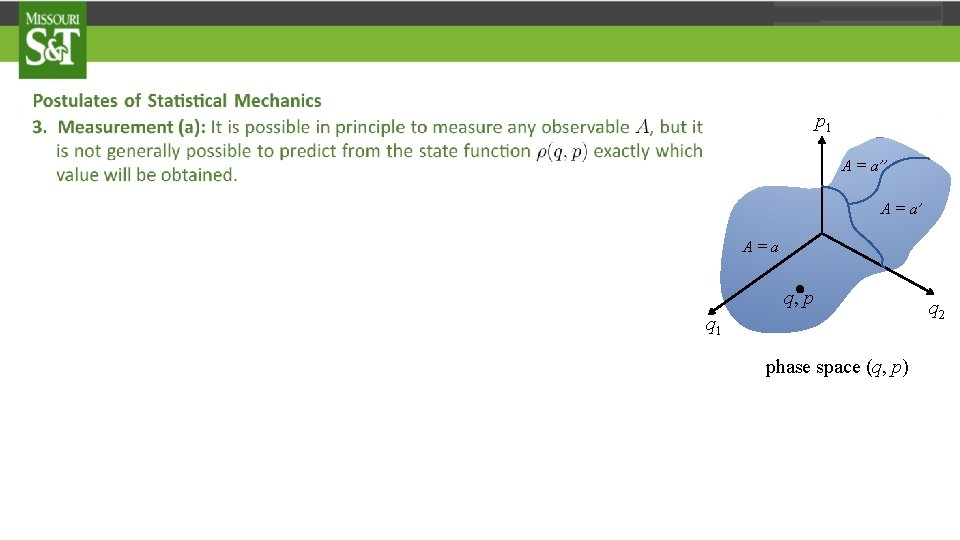p 1 A = a’’ A = a’ A=a . q, p q 1 phase space (q, p) q 2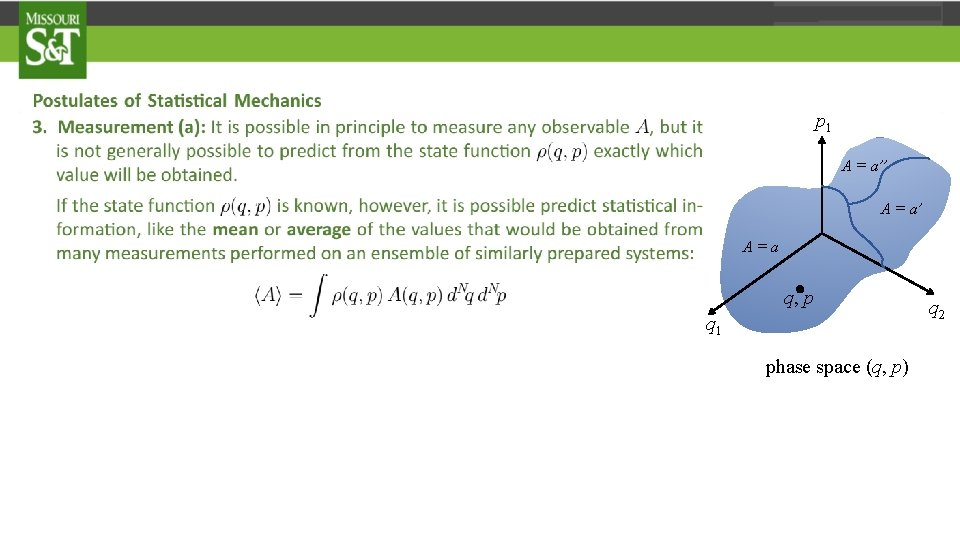p 1 A = a’’ A = a’ A=a . q, p q 1 phase space (q, p) q 2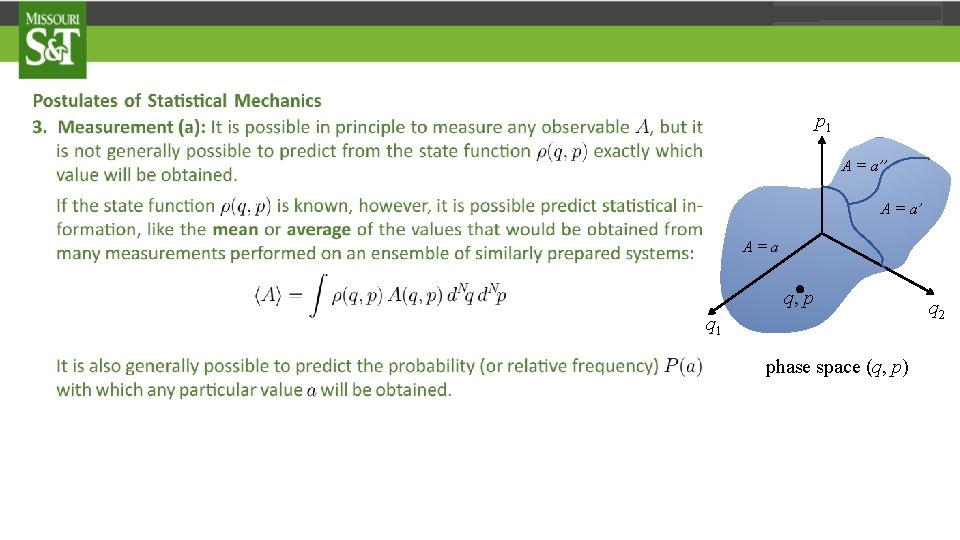p 1 A = a’’ A = a’ A=a . q, p q 1 phase space (q, p) q 2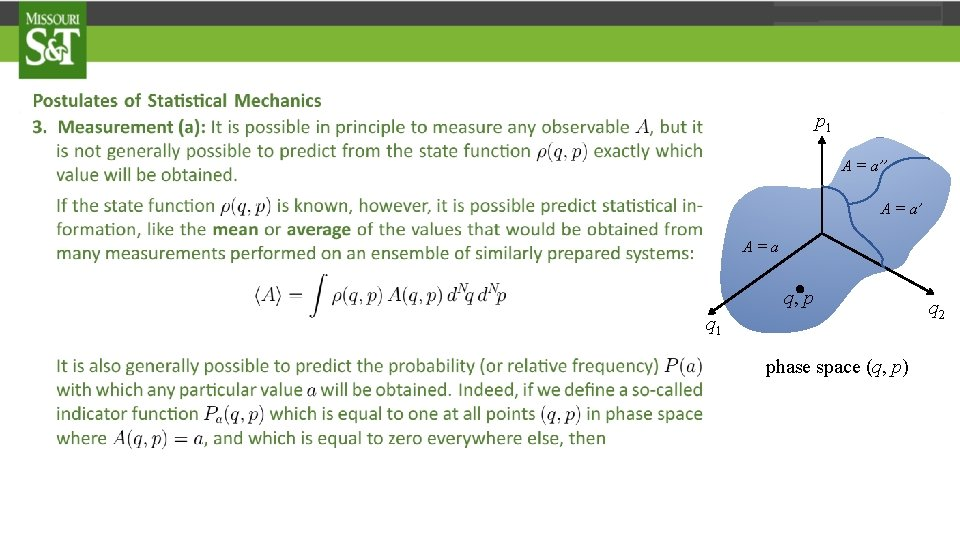p 1 A = a’’ A = a’ A=a . q, p q 1 phase space (q, p) q 2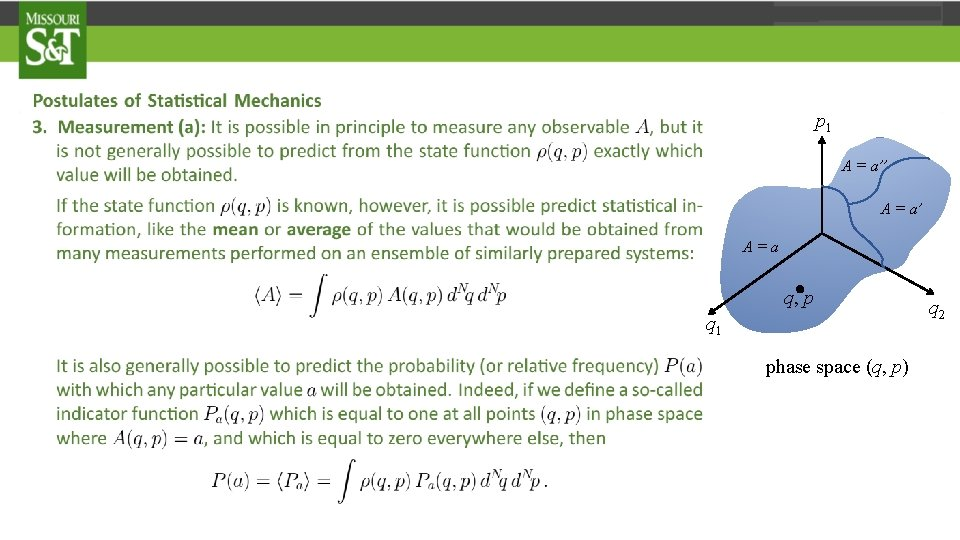p 1 A = a’’ A = a’ A=a . q, p q 1 phase space (q, p) q 2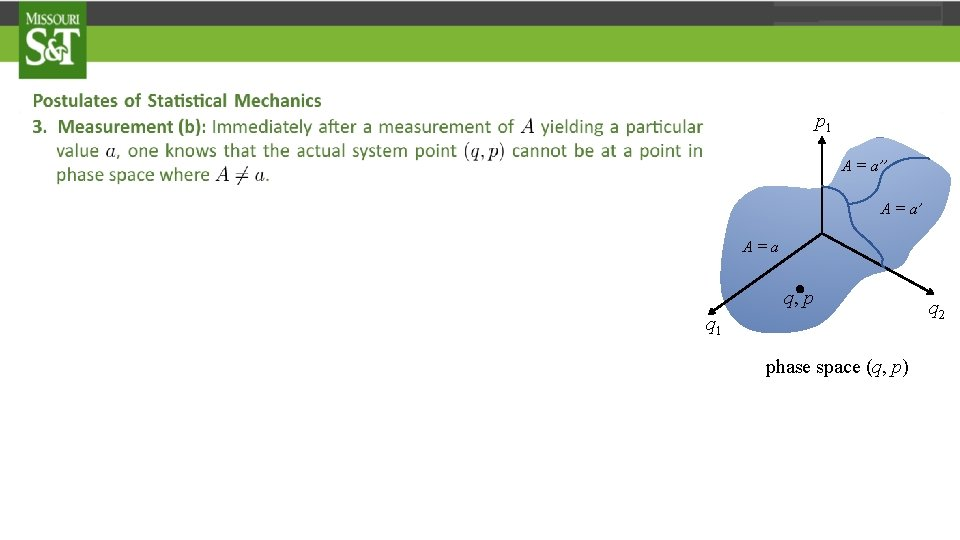p 1 A = a’’ A = a’ A=a . q, p q 1 phase space (q, p) q 2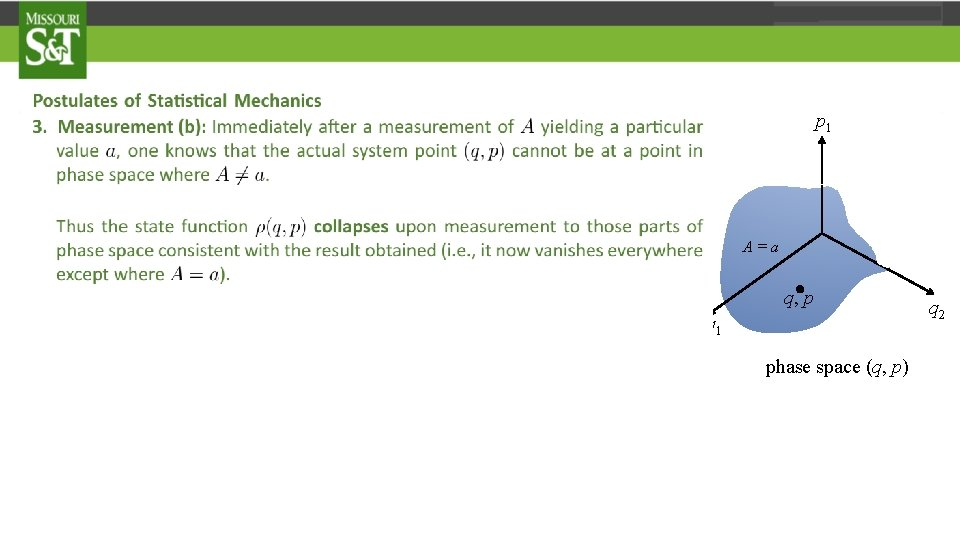p 1 A = a’’ A = a’ A=a . q, p q 1 phase space (q, p) q 2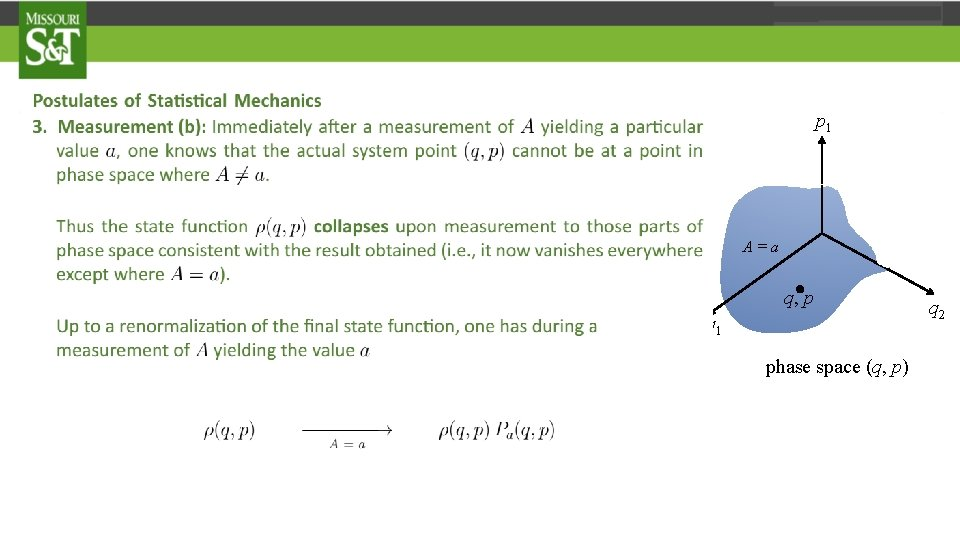p 1 A = a’’ A = a’ A=a . q, p q 1 phase space (q, p) q 2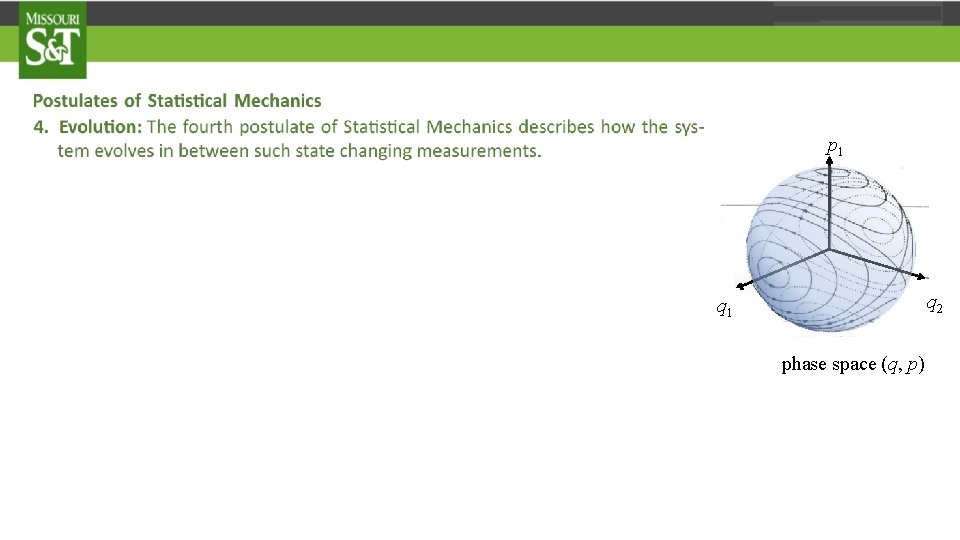p 1 q 2 q 1 phase space (q, p)p 1 q 2 q 1 phase space (q, p)p 1 q 2 q 1 phase space (q, p)The rules of Quantum Mechanics can also be stated in the form of postulates. But there aspects of Quantum Mechanics that are very odd when looked at from the point of view of classical mechanics. These odd aspects, however, have been deliberately built into the structure of theory of Quantum Mechanics to make it agree with experimental observations performed on a large (and growing) class of physical systems. So before we state the postulates, it is important to identify some of the important new features that are essential to any modern version of Quantum Mechanics. We take up this task in the next segment, after which we will state and explore the postulates for what was perhaps the first "essentially correct" version of quantum mechanics, namely, what we will refer to as Schrödinger’s wave mechanics for a single structure-less particle.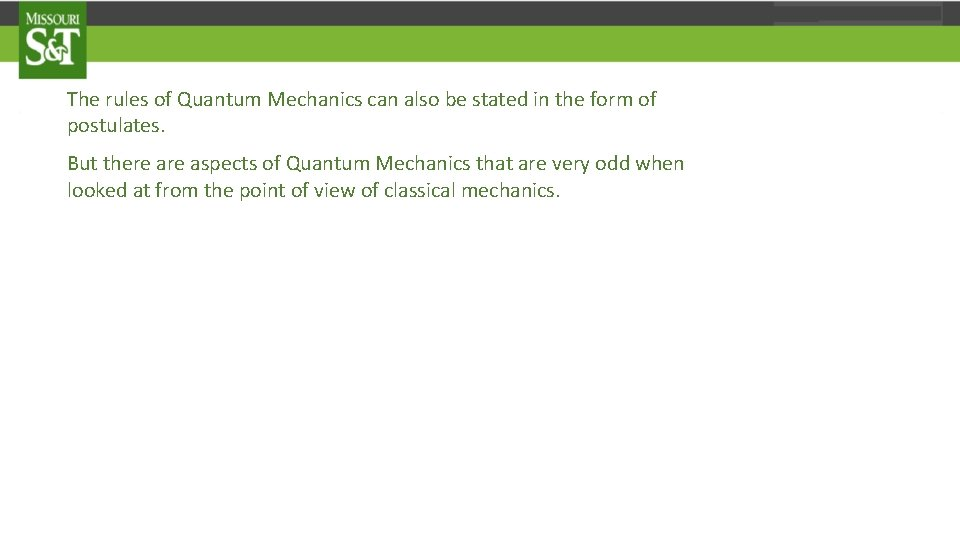The rules of Quantum Mechanics can also be stated in the form of postulates. But there aspects of Quantum Mechanics that are very odd when looked at from the point of view of classical mechanics. These odd aspects, however, have been deliberately built into the structure of theory of Quantum Mechanics to make it agree with experimental observations performed on a large (and growing) class of physical systems. So before we state the postulates, it is important to identify some of the important new features that are essential to any modern version of Quantum Mechanics. We take up this task in the next segment, after which we will state and explore the postulates for what was perhaps the first "essentially correct" version of quantum mechanics, namely, what we will refer to as Schrödinger’s wave mechanics for a single structure-less particle.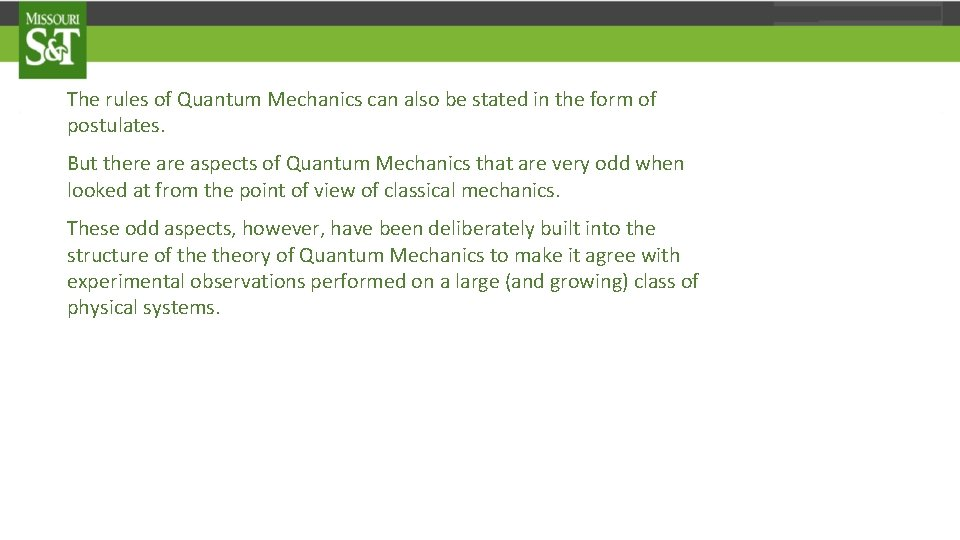The rules of Quantum Mechanics can also be stated in the form of postulates. But there aspects of Quantum Mechanics that are very odd when looked at from the point of view of classical mechanics. These odd aspects, however, have been deliberately built into the structure of theory of Quantum Mechanics to make it agree with experimental observations performed on a large (and growing) class of physical systems. So before we state the postulates, it is important to identify some of the important new features that are essential to any modern version of Quantum Mechanics. We take up this task in the next segment, after which we will state and explore the postulates for what was perhaps the first "essentially correct" version of quantum mechanics, namely, what we will refer to as Schrödinger’s wave mechanics for a single structure-less particle.The rules of Quantum Mechanics can also be stated in the form of postulates. But there aspects of Quantum Mechanics that are very odd when looked at from the point of view of classical mechanics. These odd aspects, however, have been deliberately built into the structure of theory of Quantum Mechanics to make it agree with experimental observations performed on a large (and growing) class of physical systems. So before we state the postulates, it is important to identify some of the important new features that are essential to any modern version of Quantum Mechanics. We take up this task in the next segment, after which we will state and explore the postulates for what was perhaps the first "essentially correct" version of quantum mechanics, namely, what we will refer to as Schrödinger’s wave mechanics for a single structure-less particle.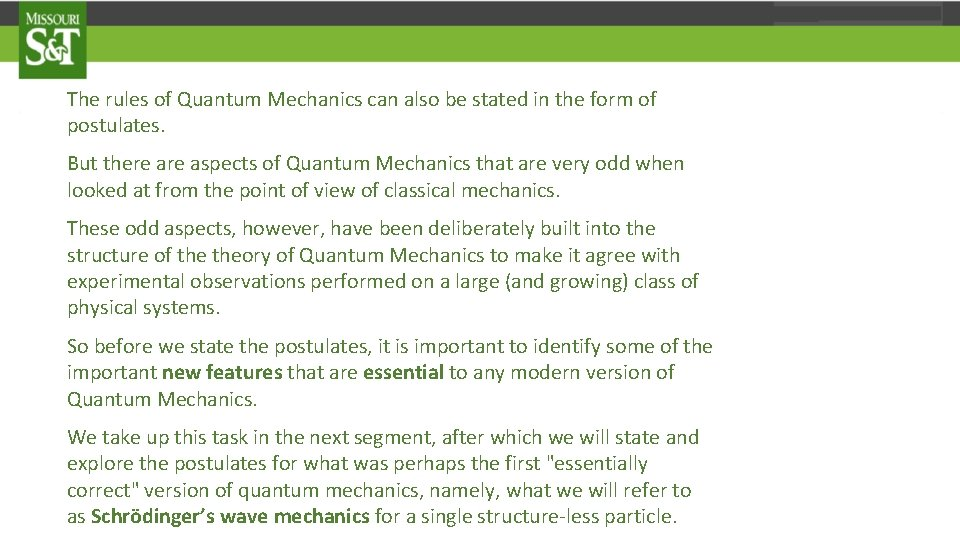The rules of Quantum Mechanics can also be stated in the form of postulates. But there aspects of Quantum Mechanics that are very odd when looked at from the point of view of classical mechanics. These odd aspects, however, have been deliberately built into the structure of theory of Quantum Mechanics to make it agree with experimental observations performed on a large (and growing) class of physical systems. So before we state the postulates, it is important to identify some of the important new features that are essential to any modern version of Quantum Mechanics. We take up this task in the next segment, after which we will state and explore the postulates for what was perhaps the first "essentially correct" version of quantum mechanics, namely, what we will refer to as Schrödinger’s wave mechanics for a single structure-less particle.